# RD Sharma Solutions for Class 9 Maths Chapter 16 Circles

## RD Sharma Solutions Class 9 Maths Chapter 16 – Free PDF Download

RD Sharma Solutions Class 9 Maths Chapter 16 Circles are provided here in PDF format for an easy understanding of concepts. In brief, a circle is a closed plane curve consisting of all points at a given distance from a fixed point within it, which is called the center of the circle. The center of a circle always lies in the interior of the circle. The distance between any points of the circumference of the circle and the center of the circle is called the radius. Also, the longest chord of a circle is its diameter. RD Sharma Solutions help students to practice questions based on different concepts such as radius, segment, sector and diameter of circle.

Check the detailed RD Sharma Solutions for Class 9 Maths Chapter 16 and score good marks in exams. The comprehensive solutions to the exercise questions can be checked from the following link. The solutions are designed by expert teachers as per the latest CBSE syllabus updated for 2022-23.

## Download PDF of RD Sharma Solutions for Class 9 Maths Chapter 16 Circles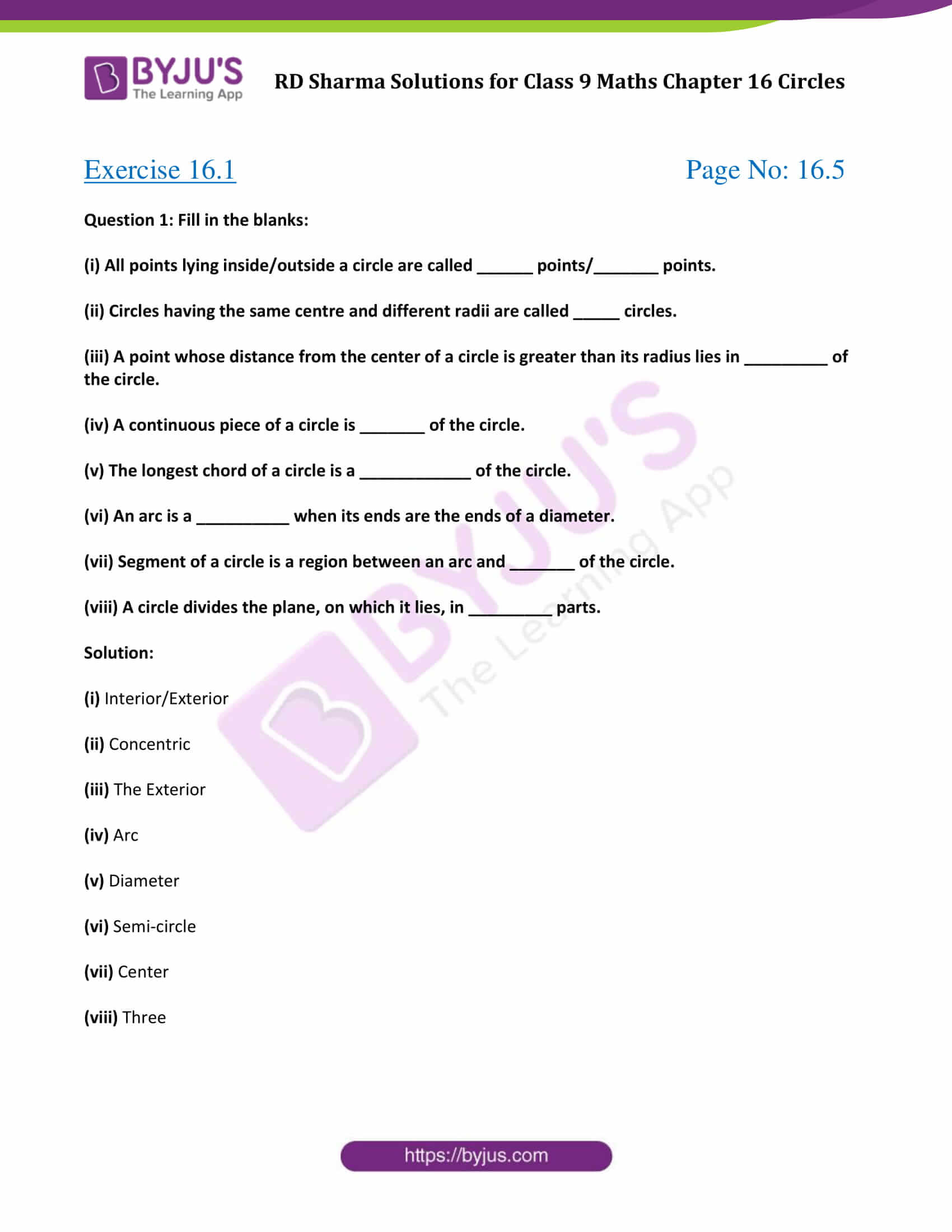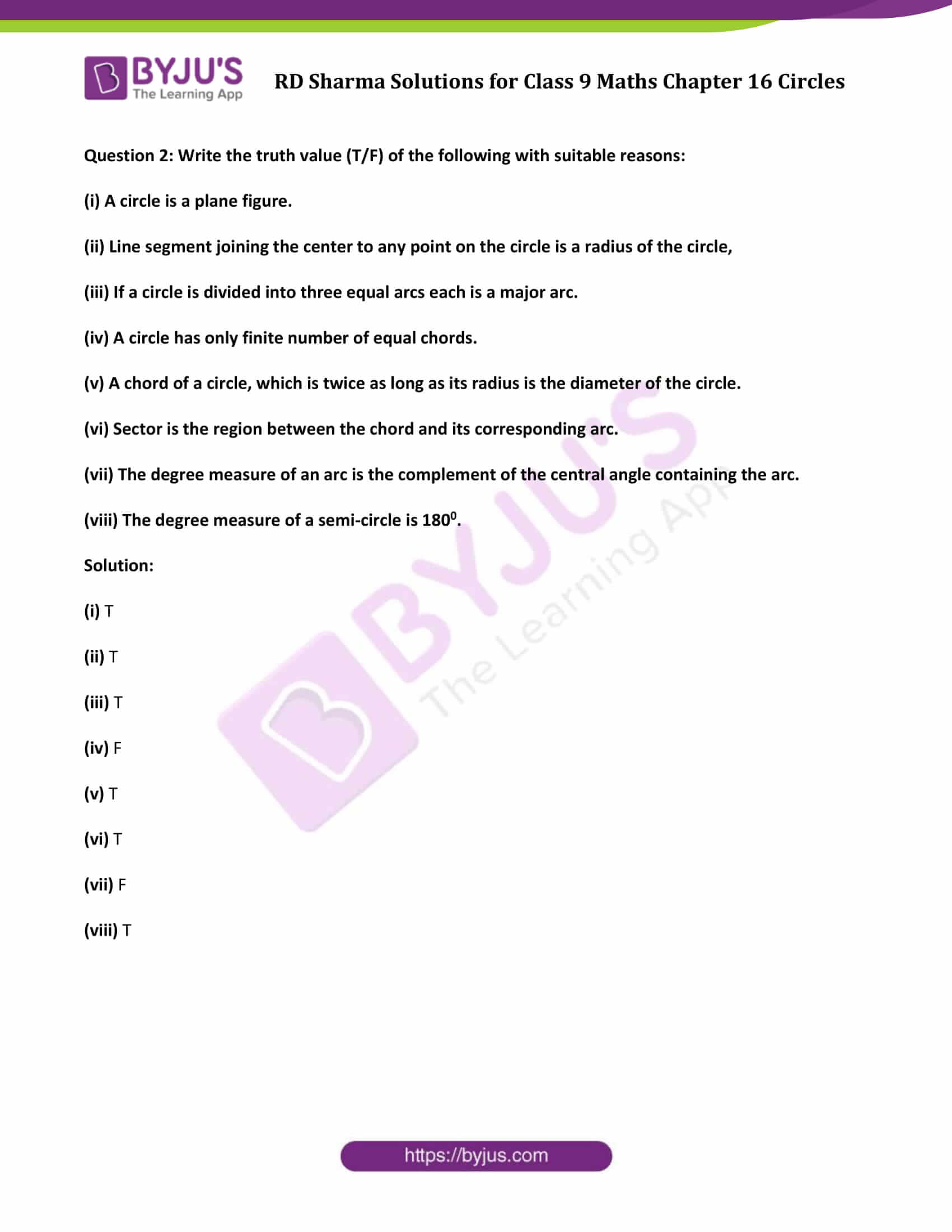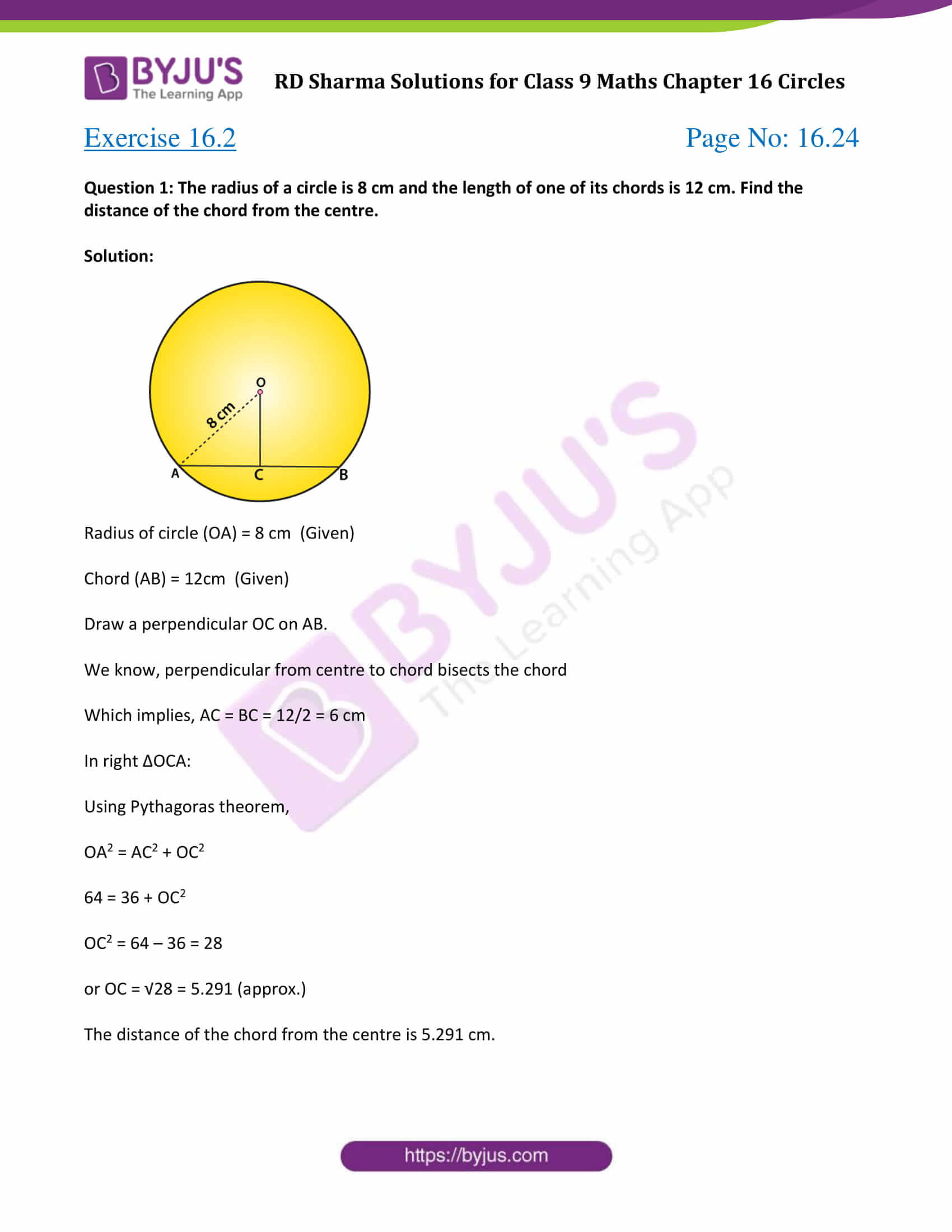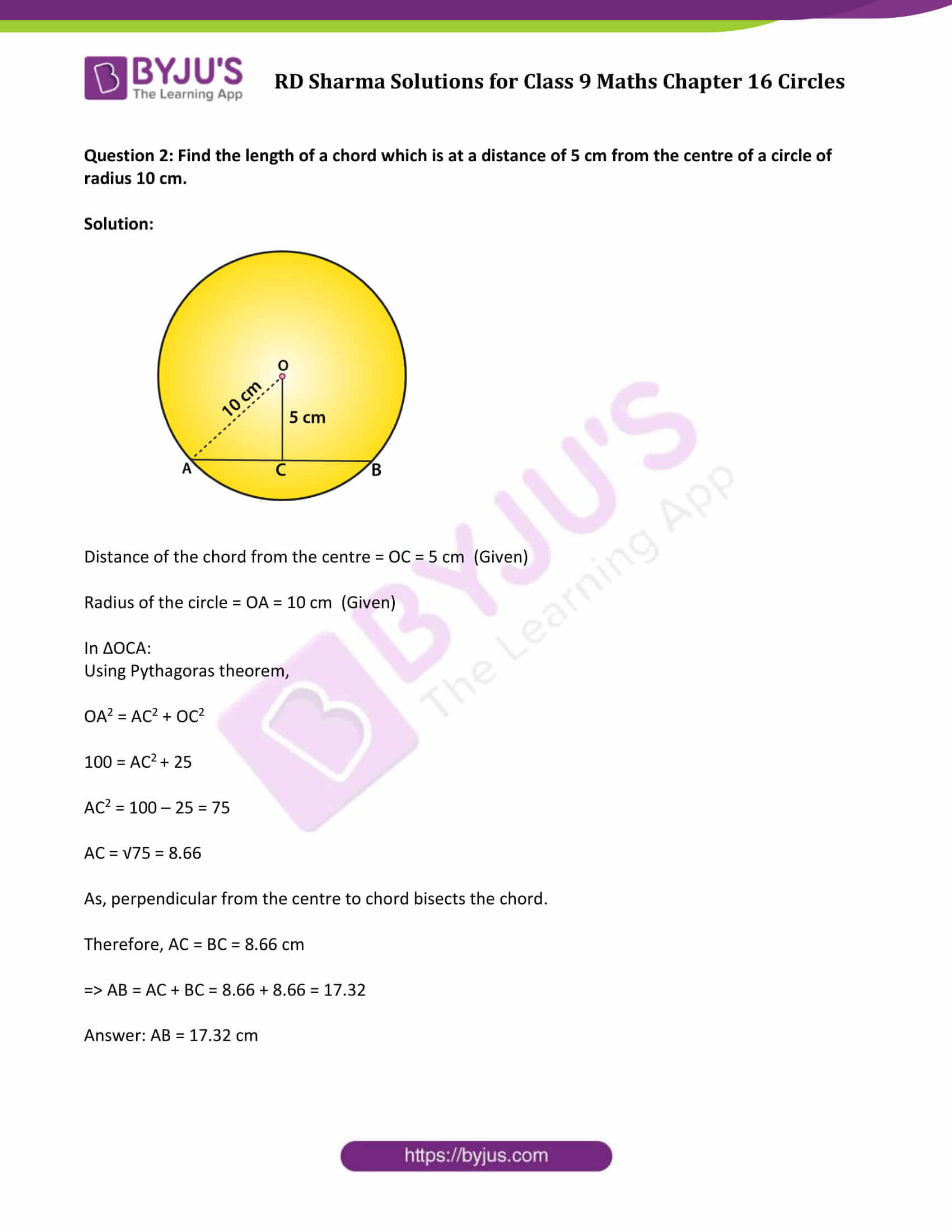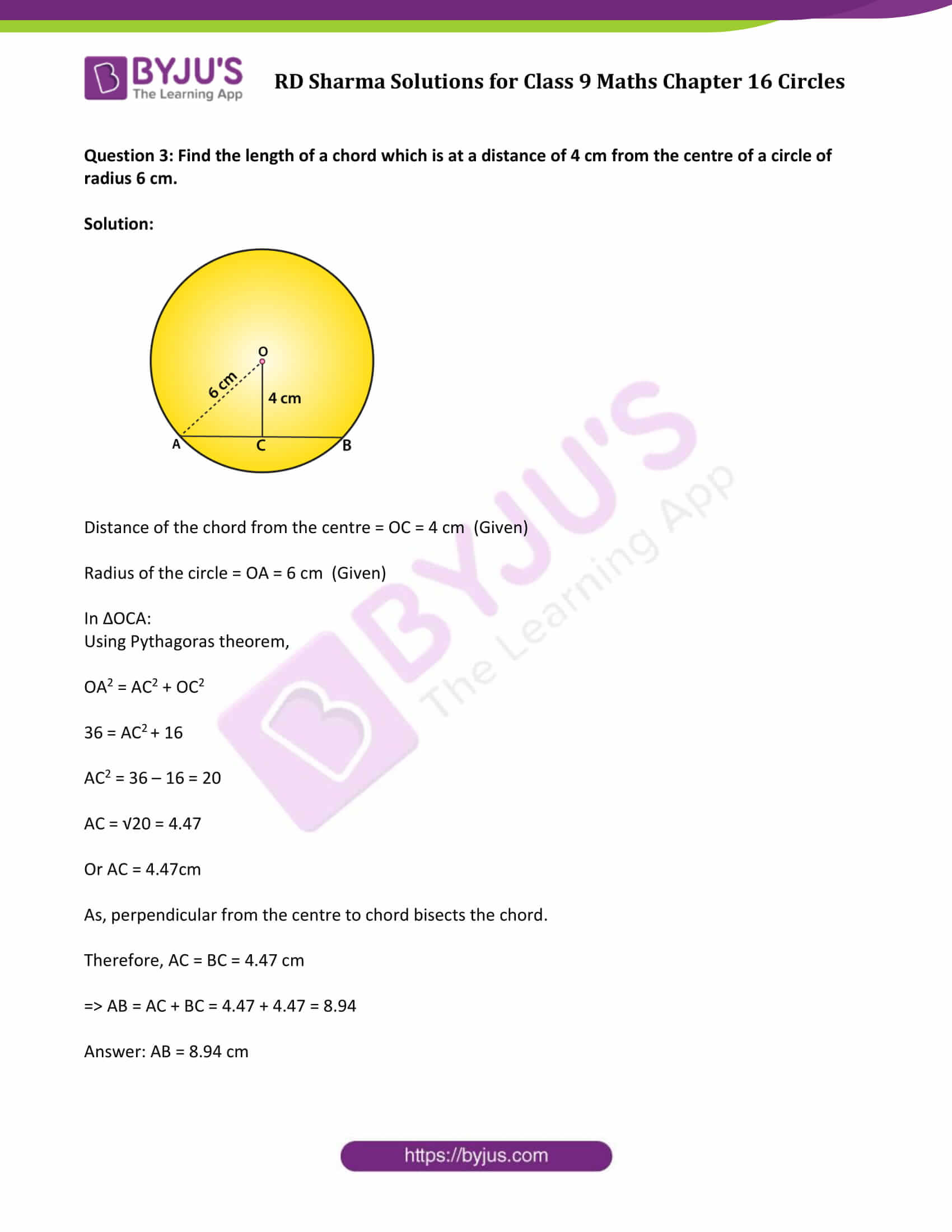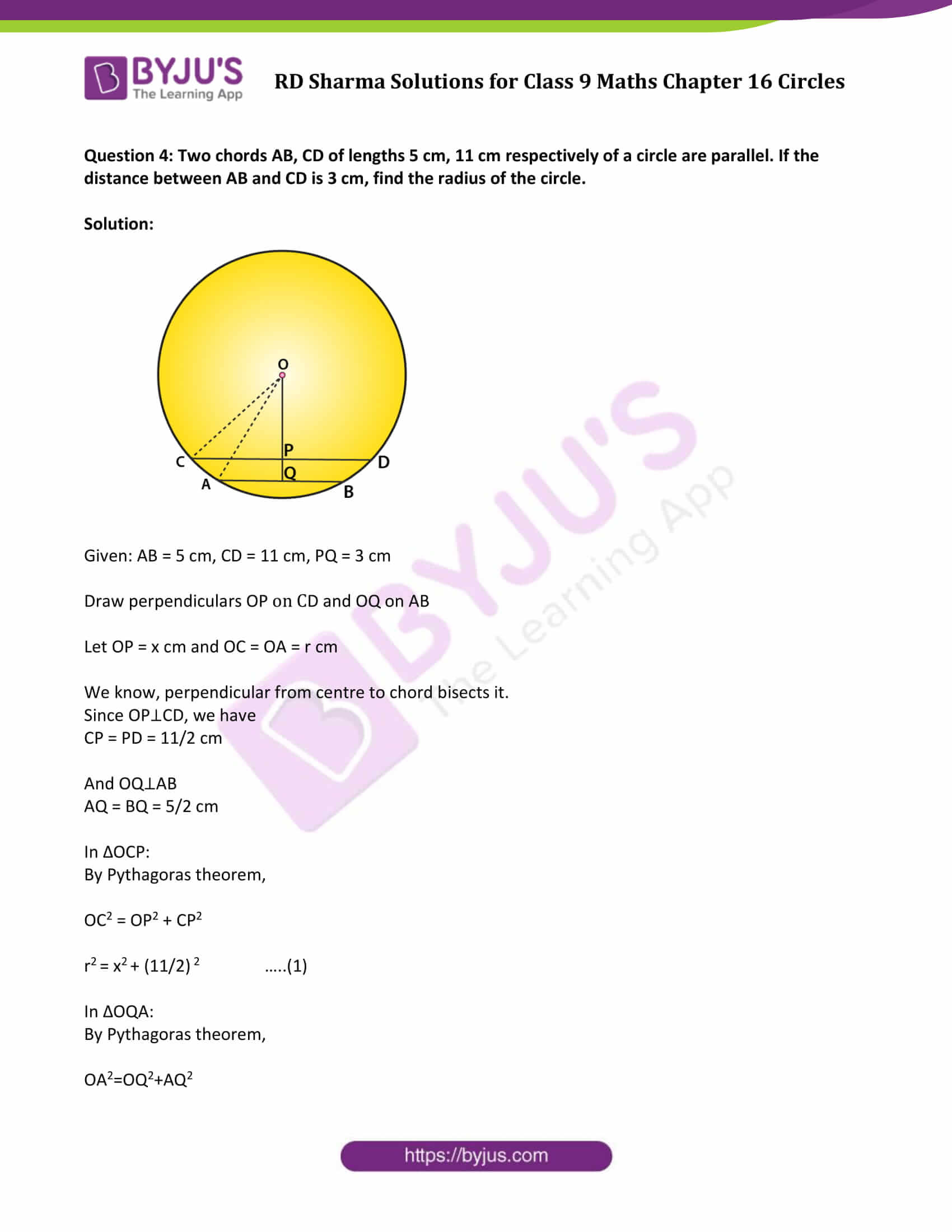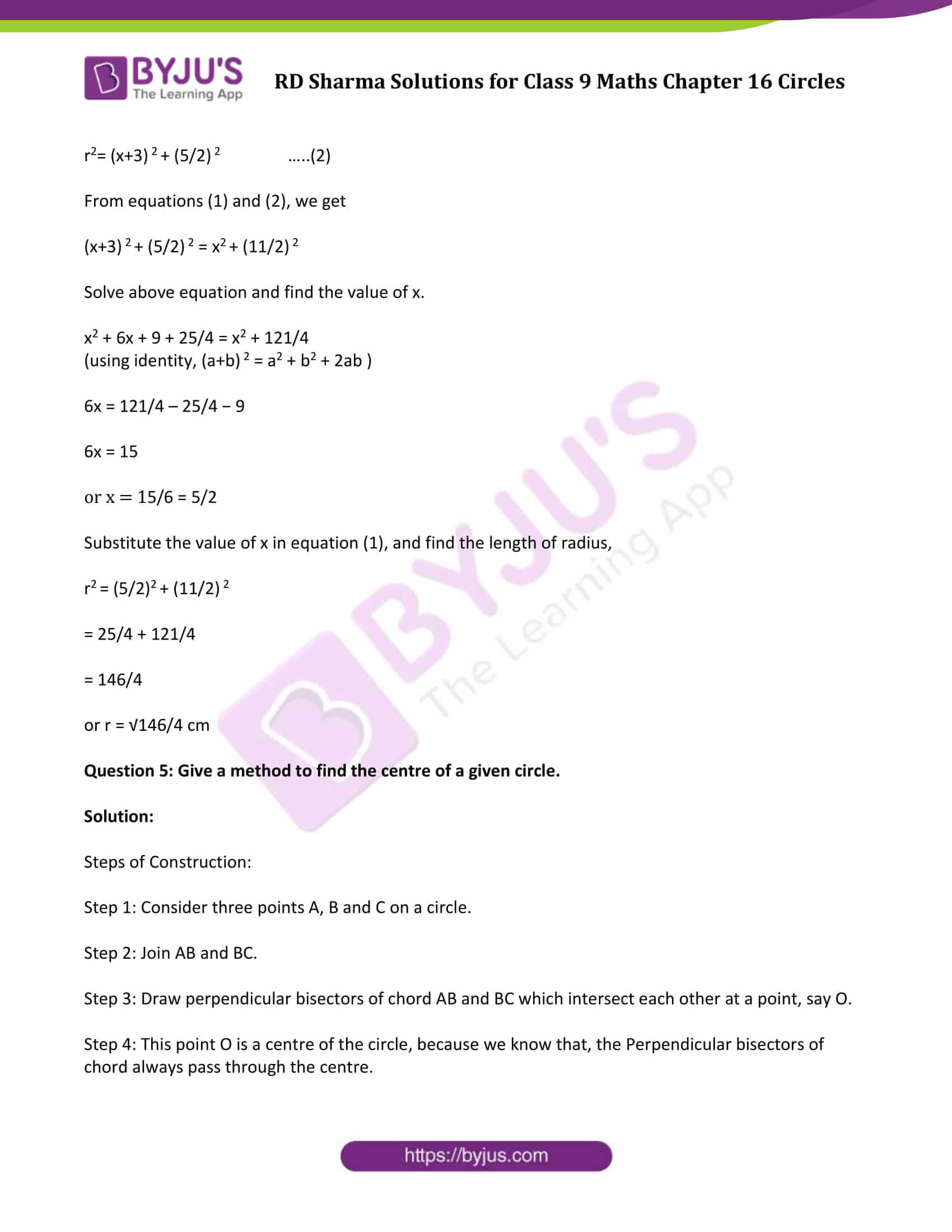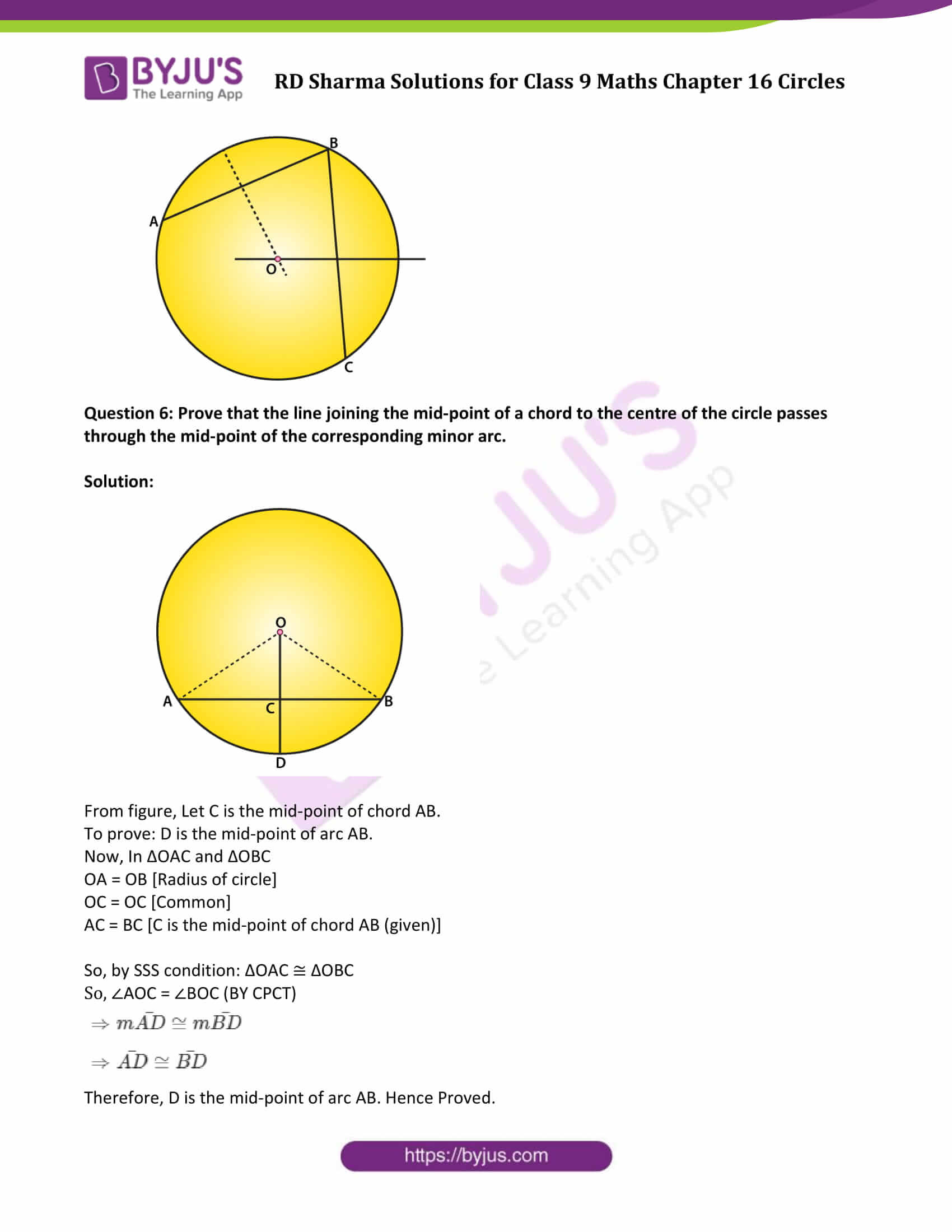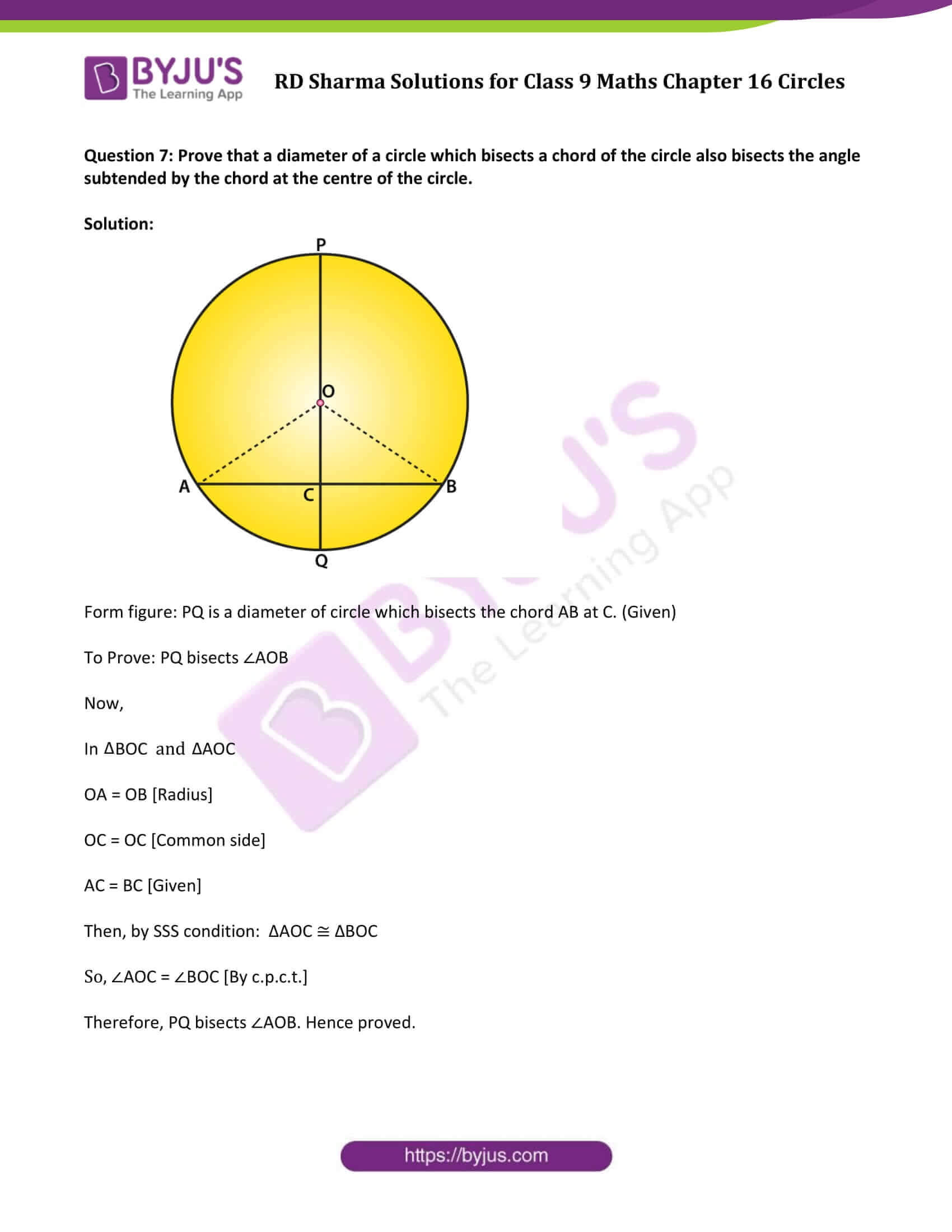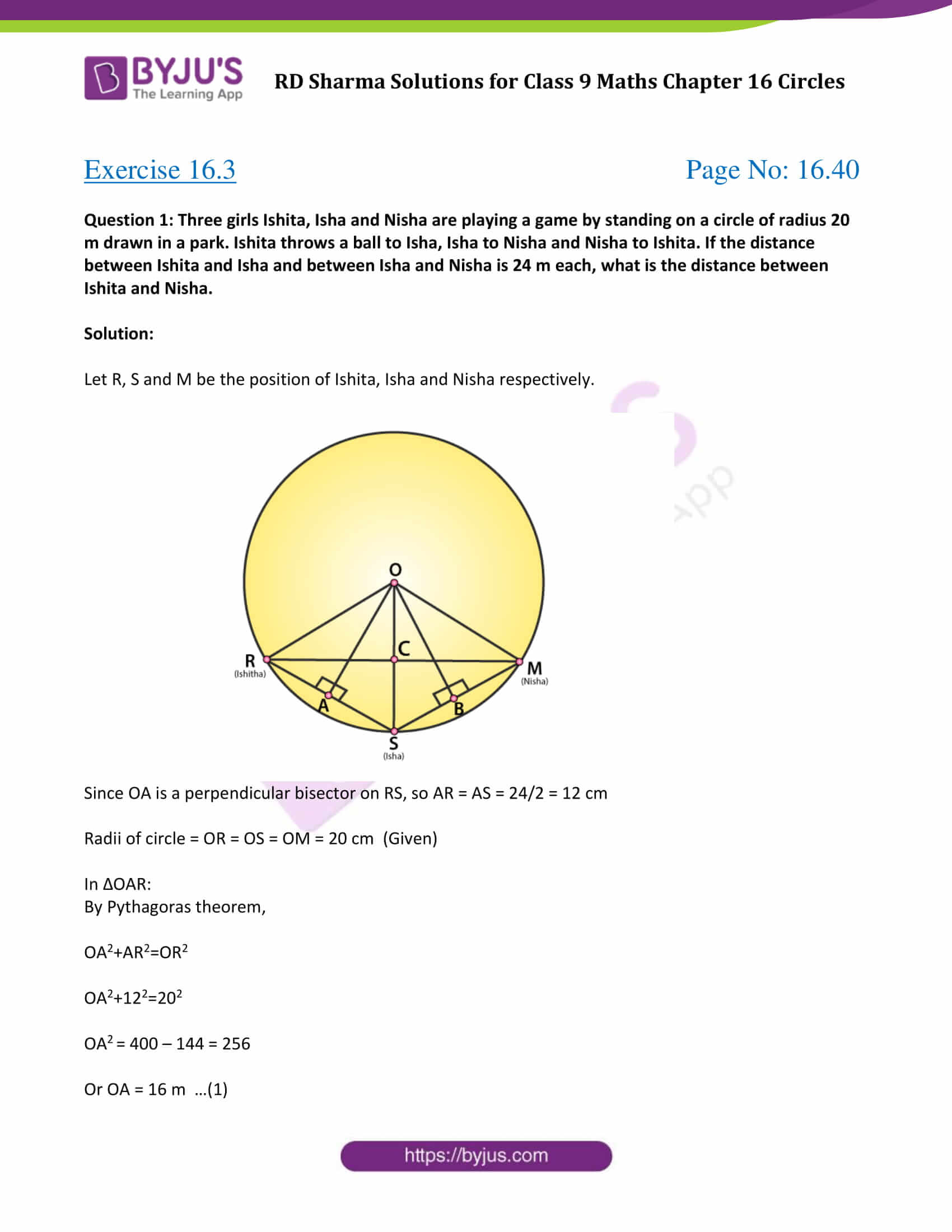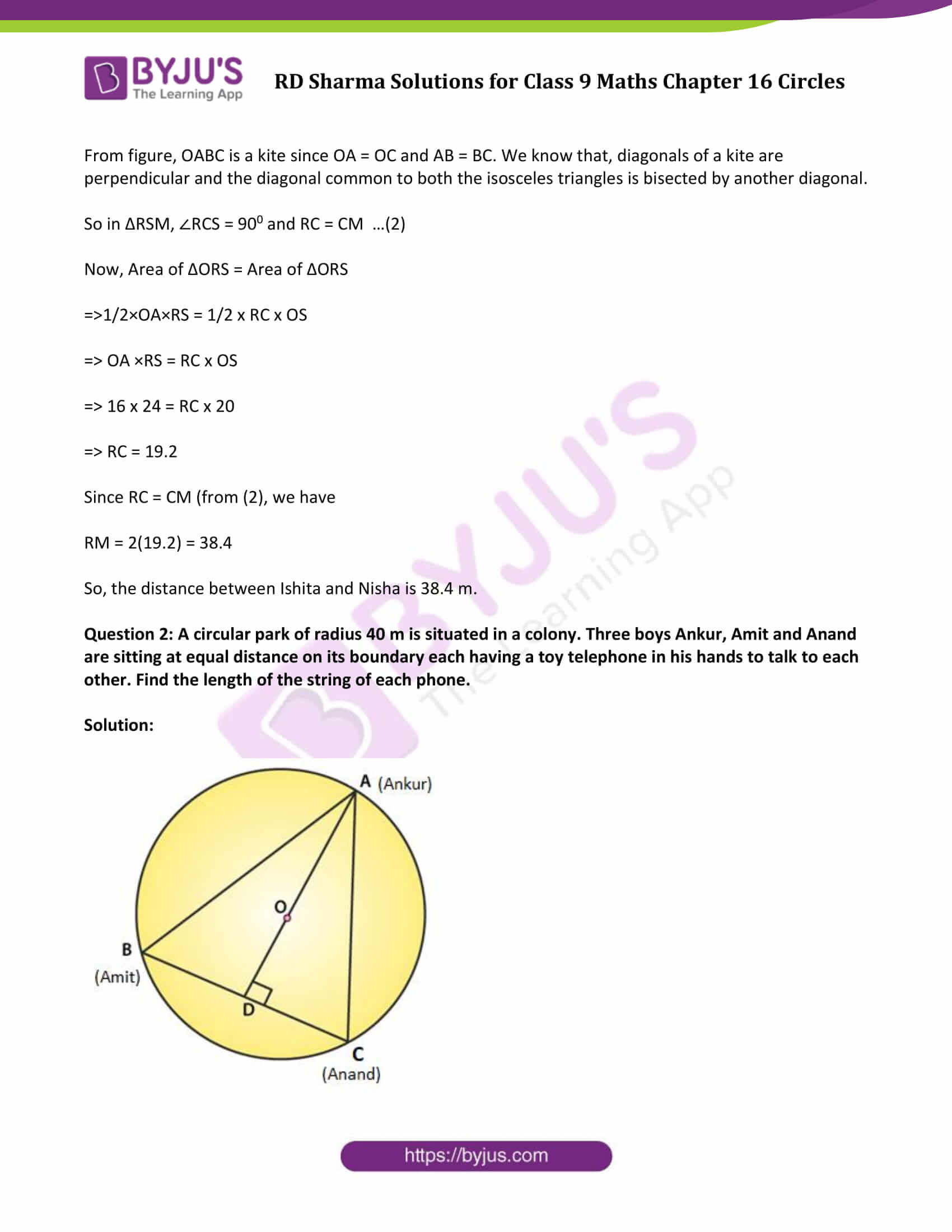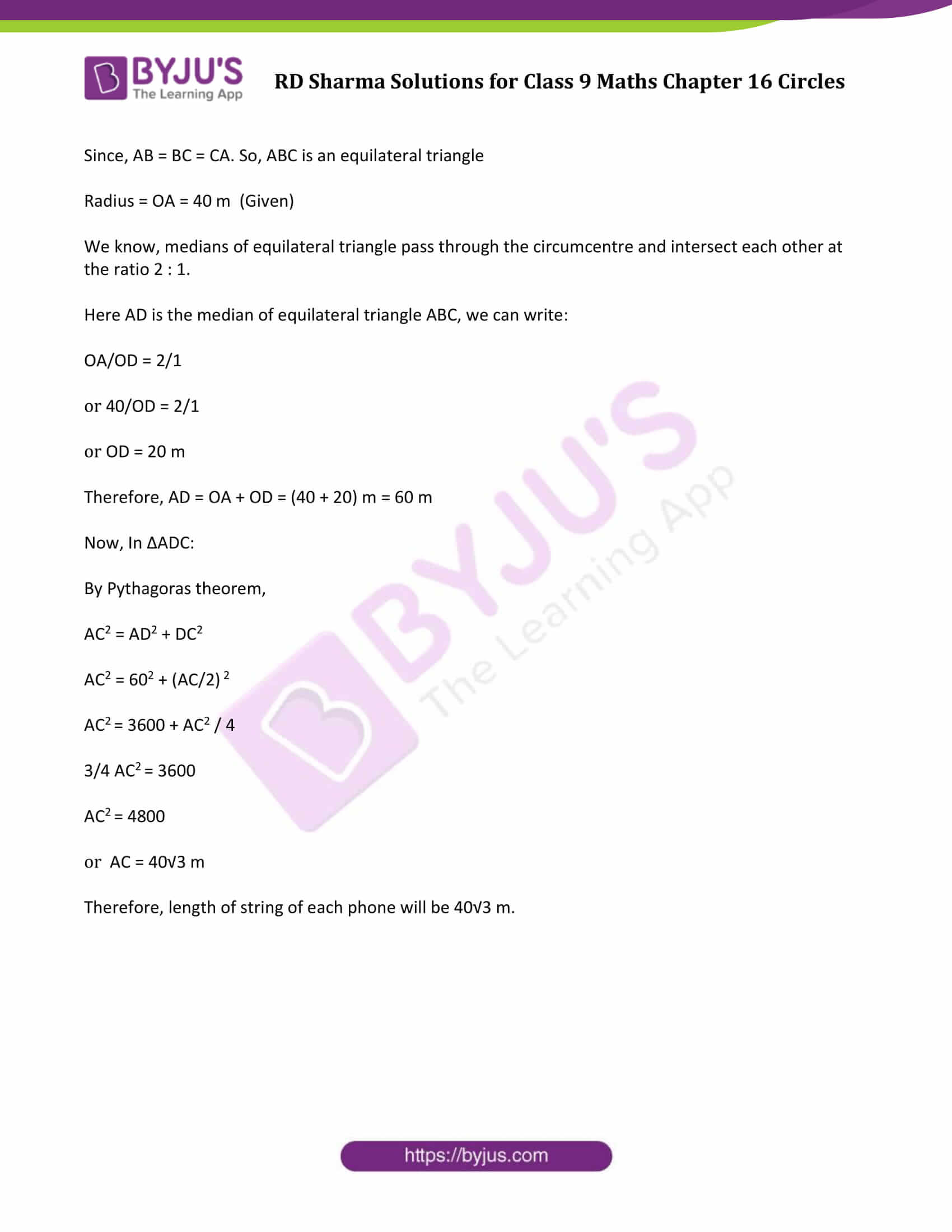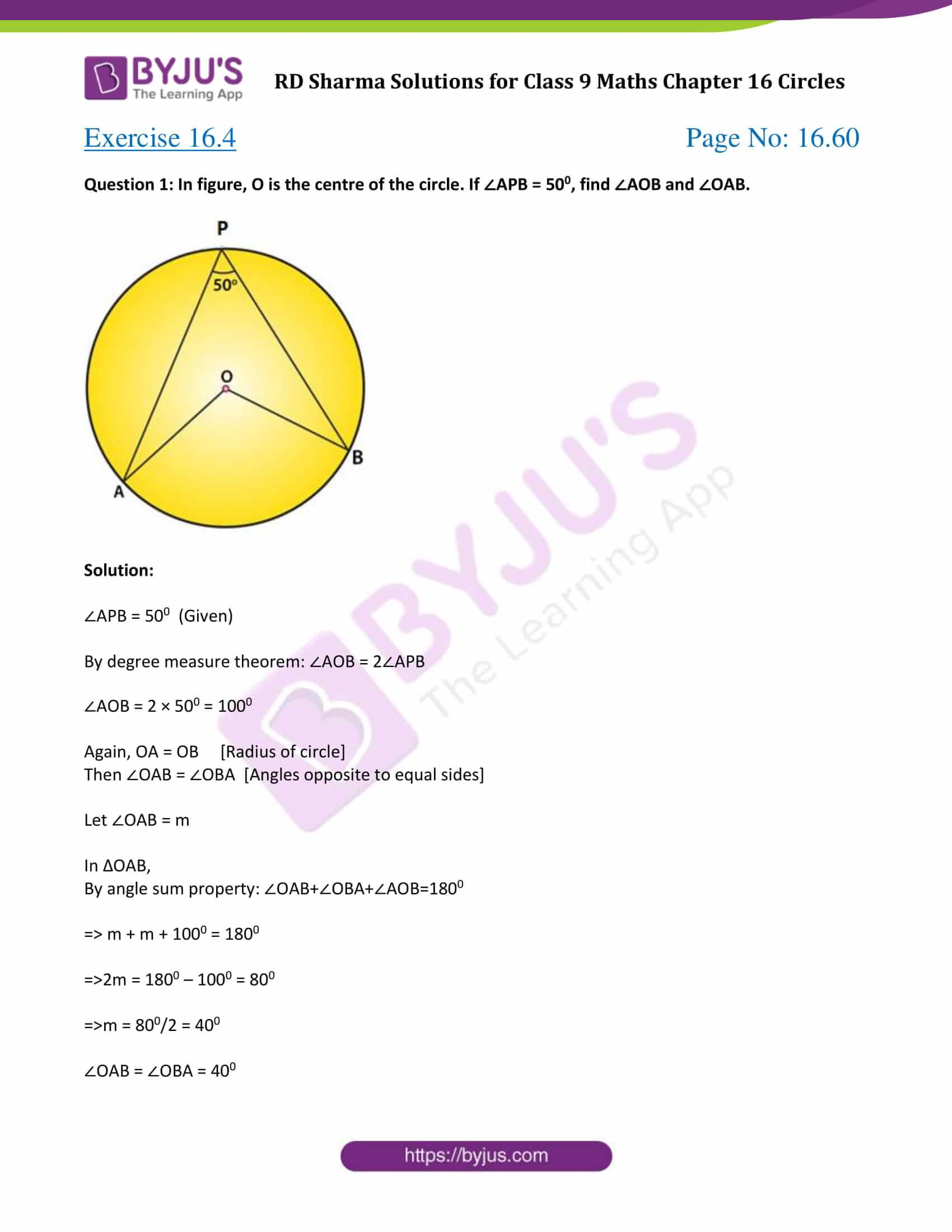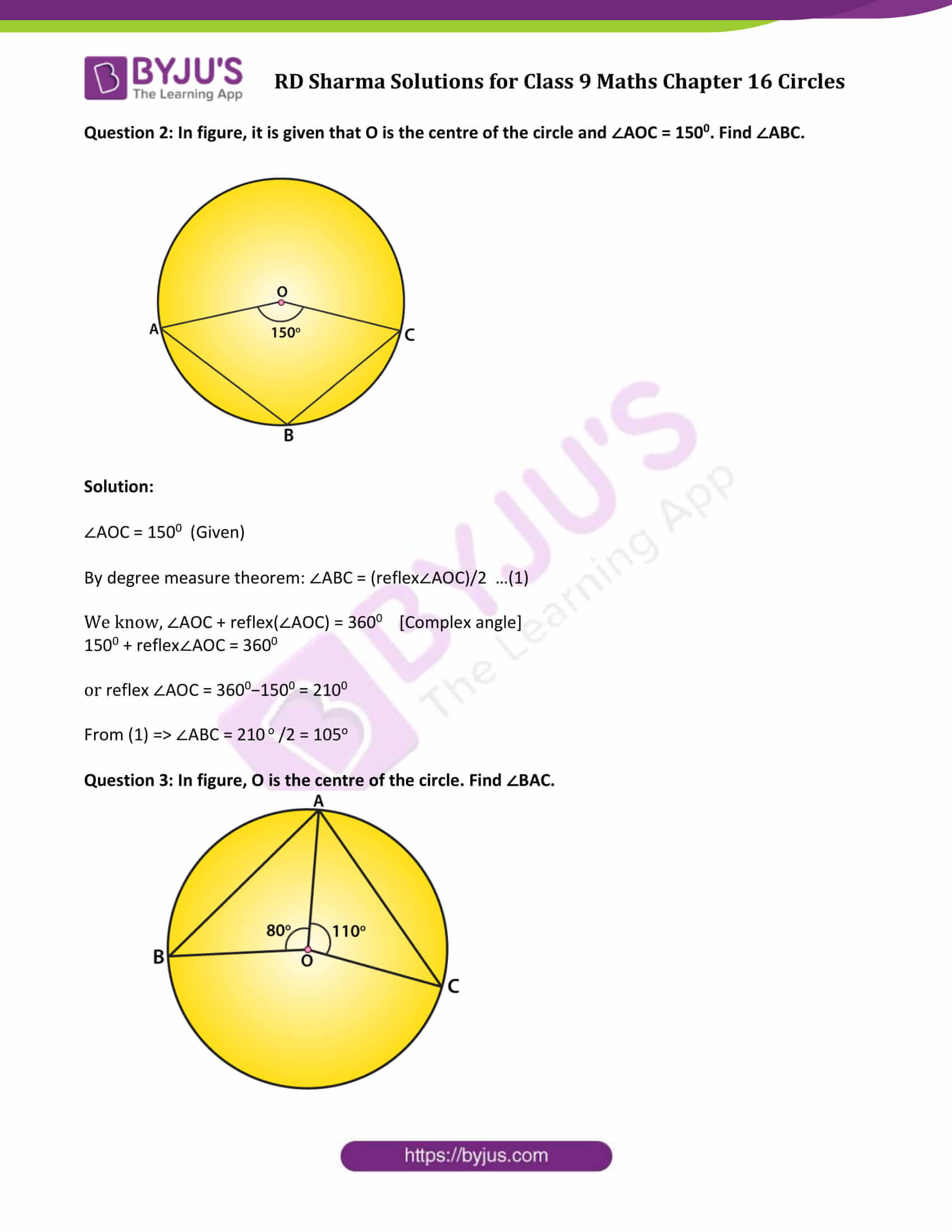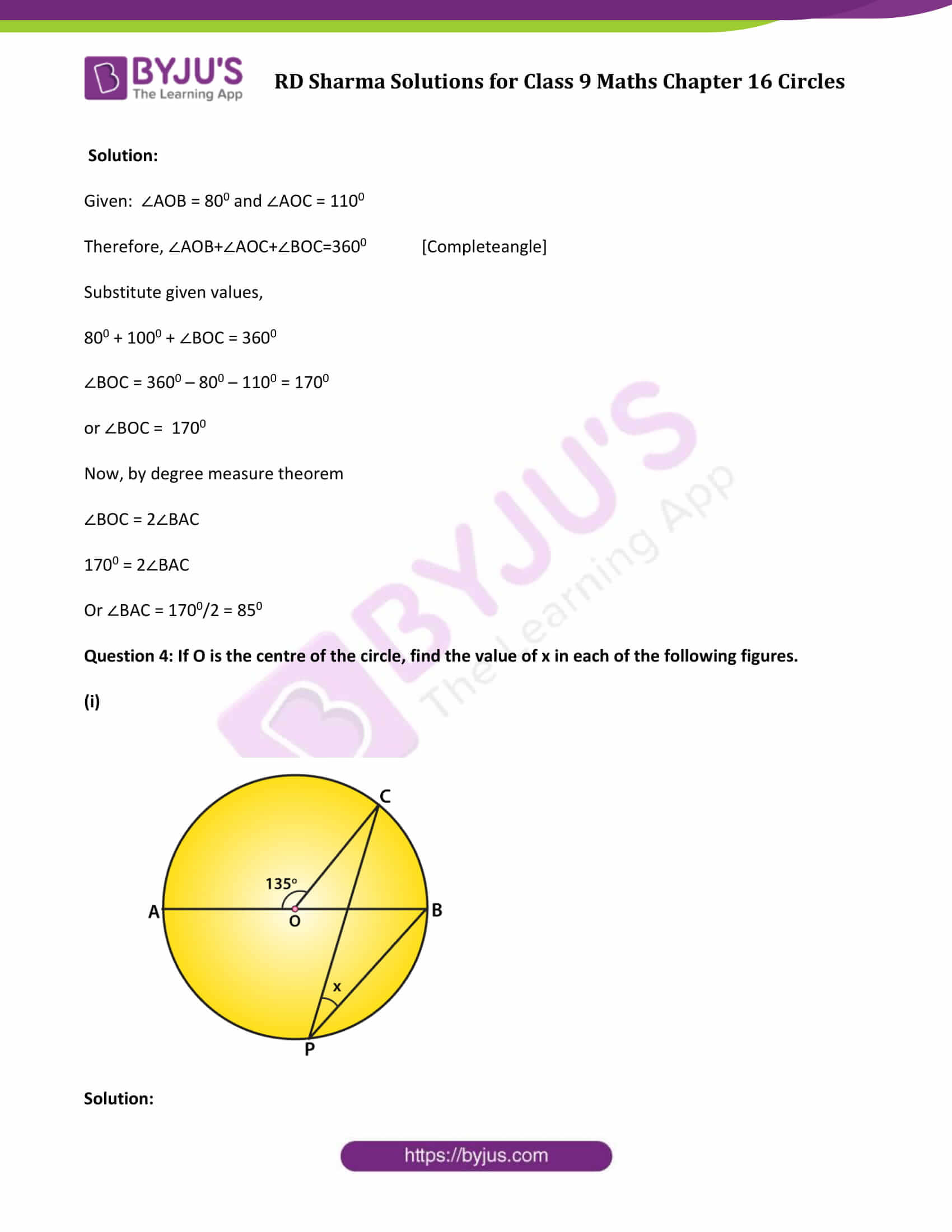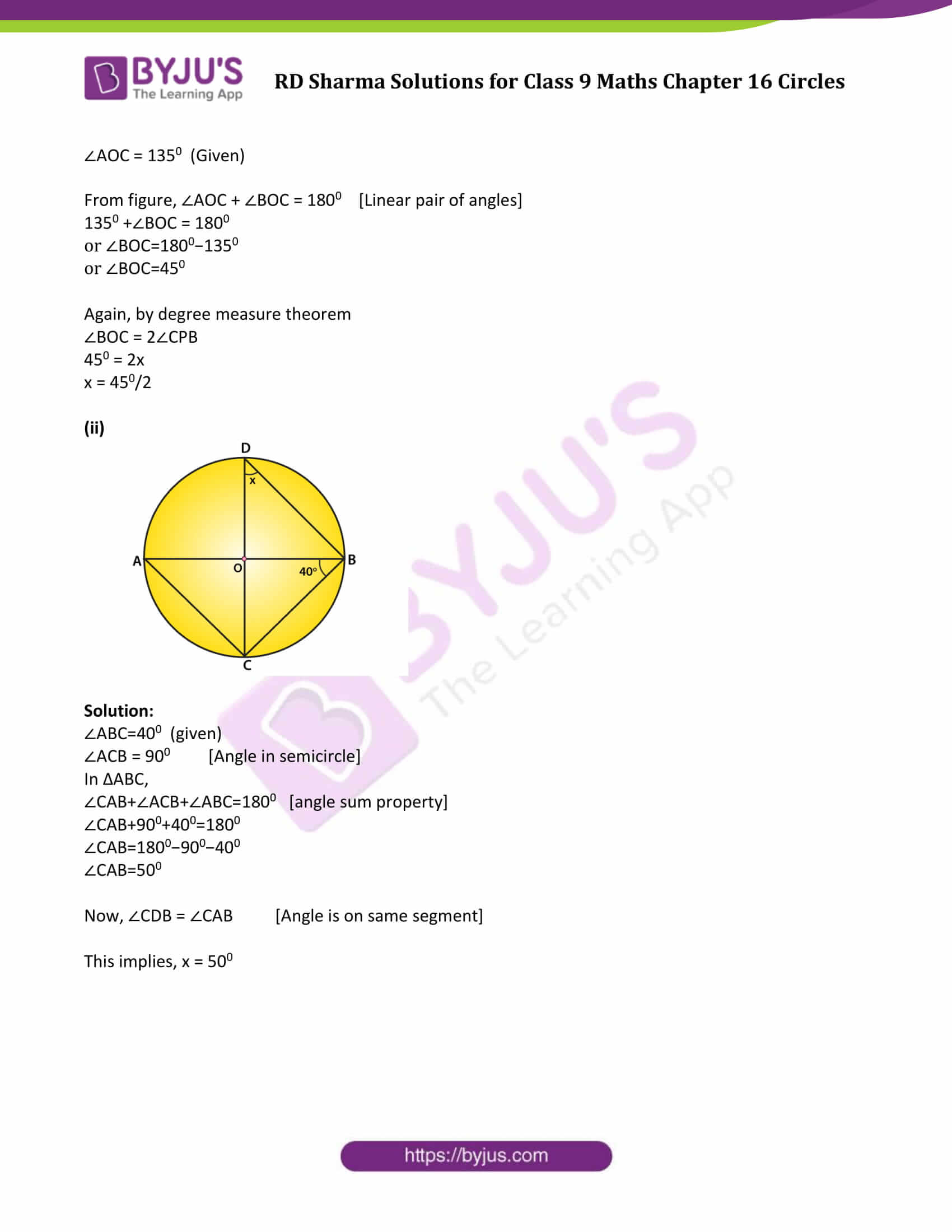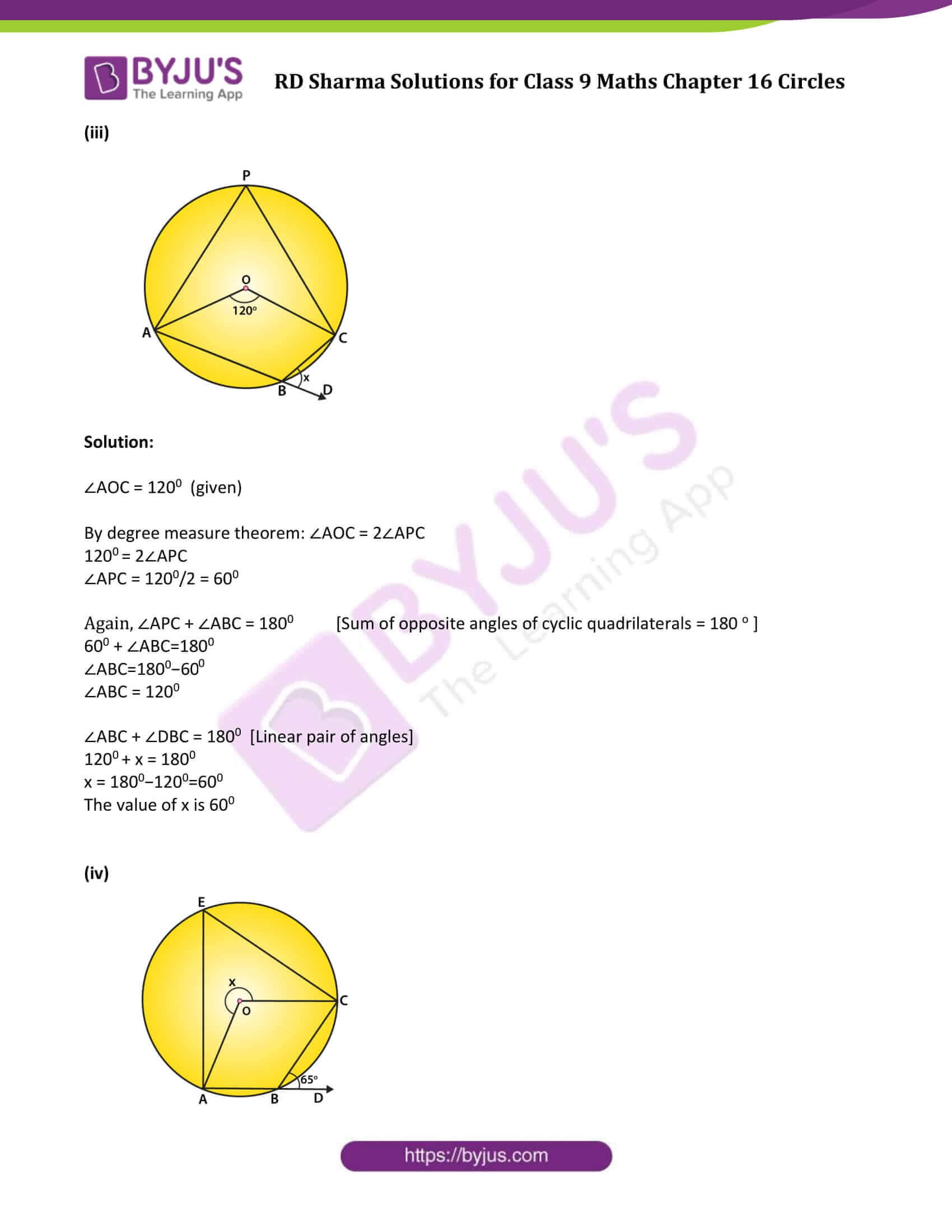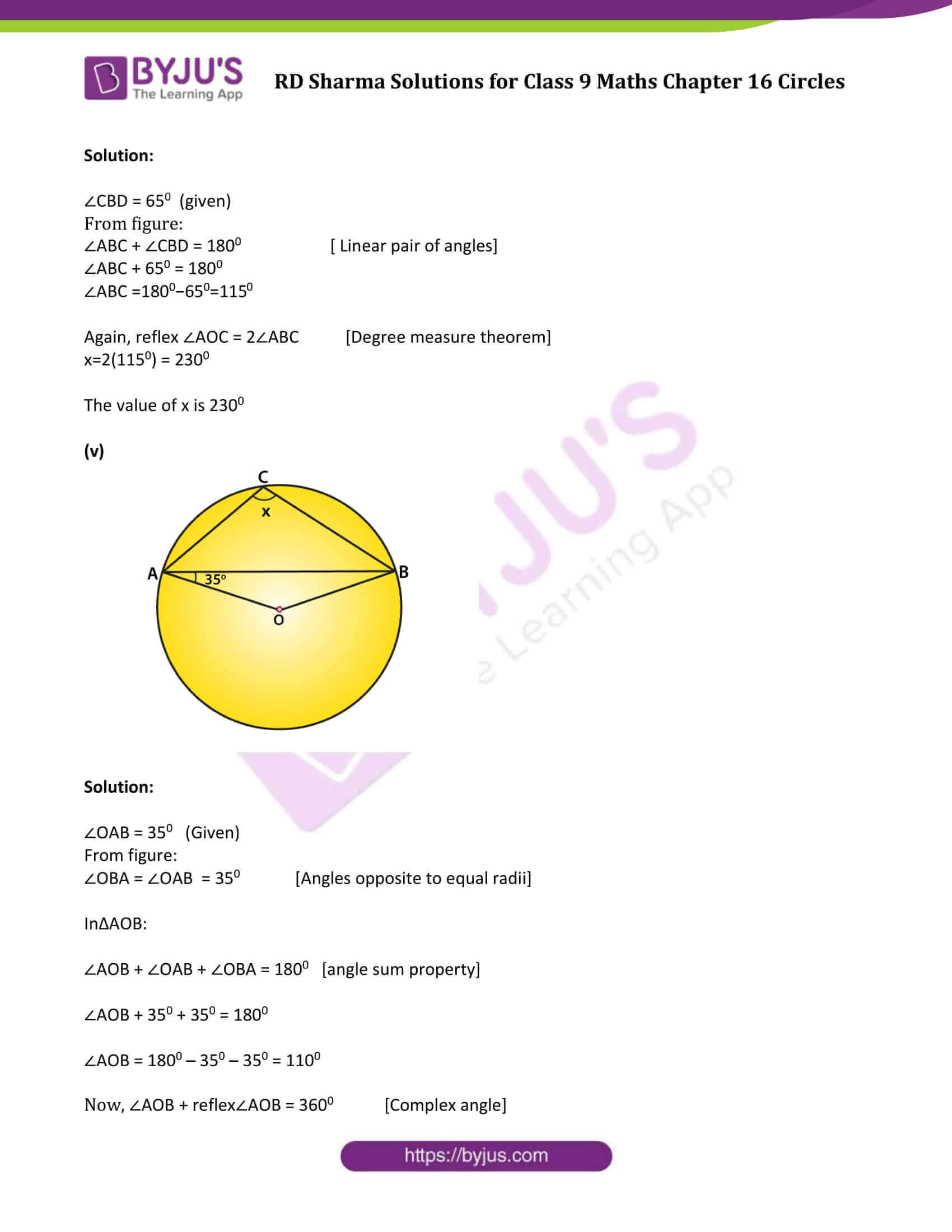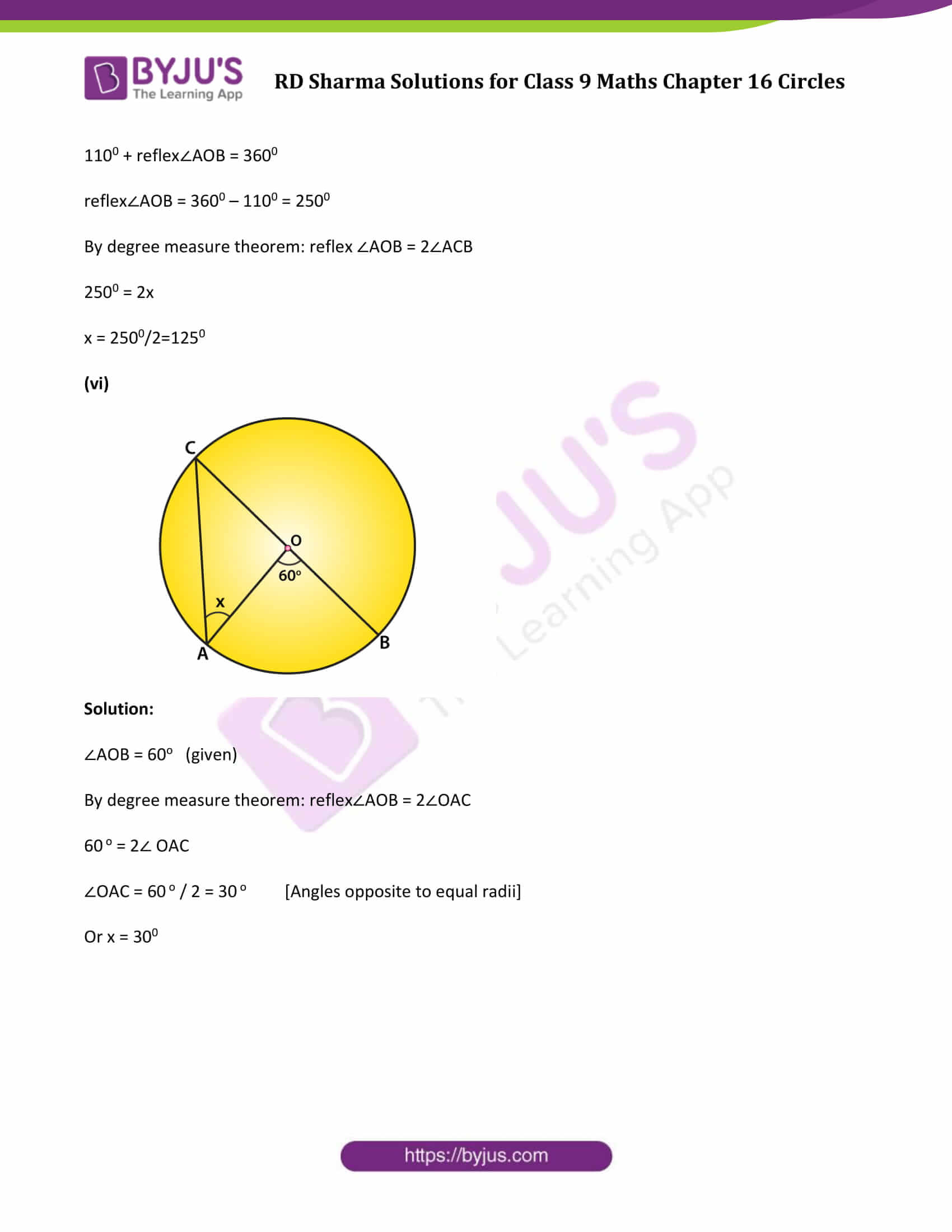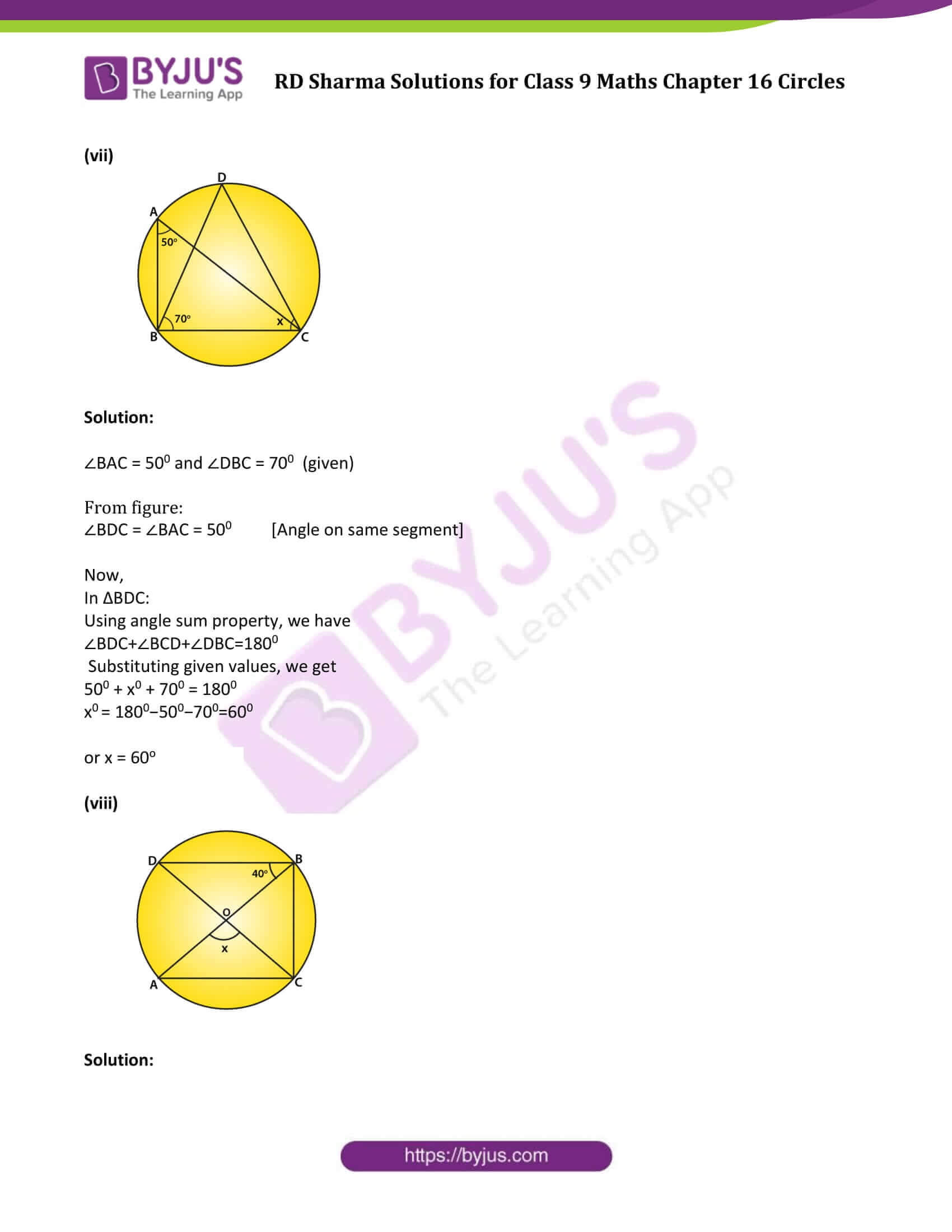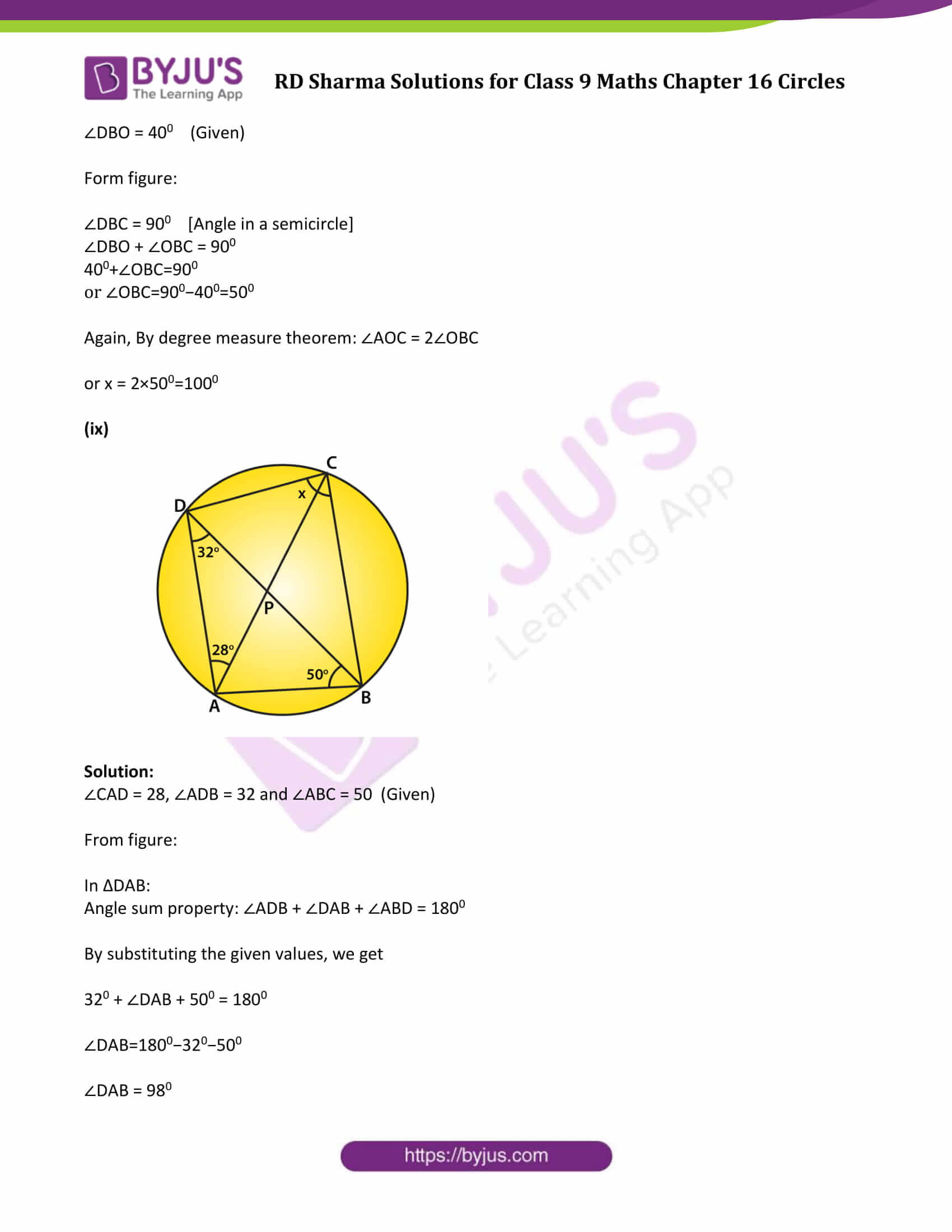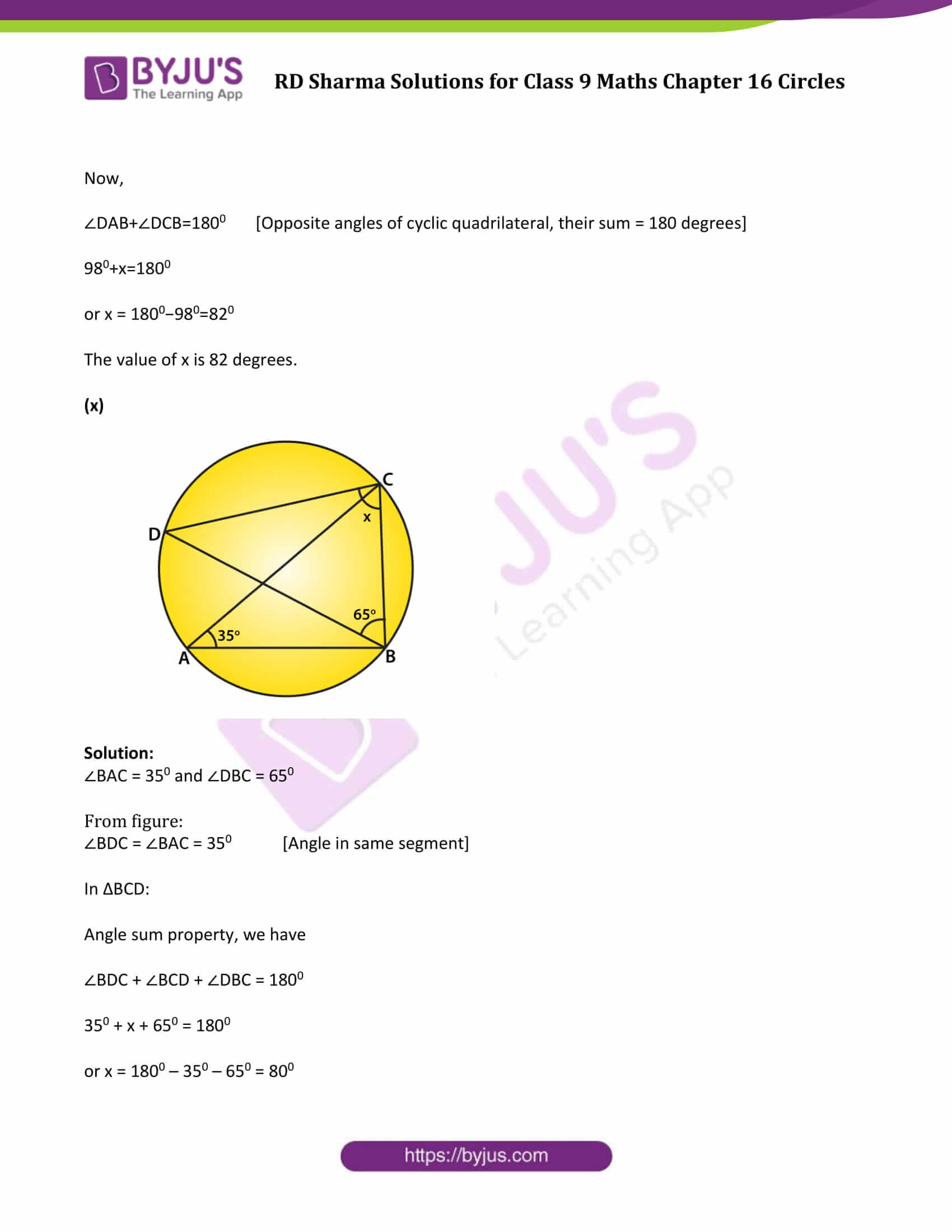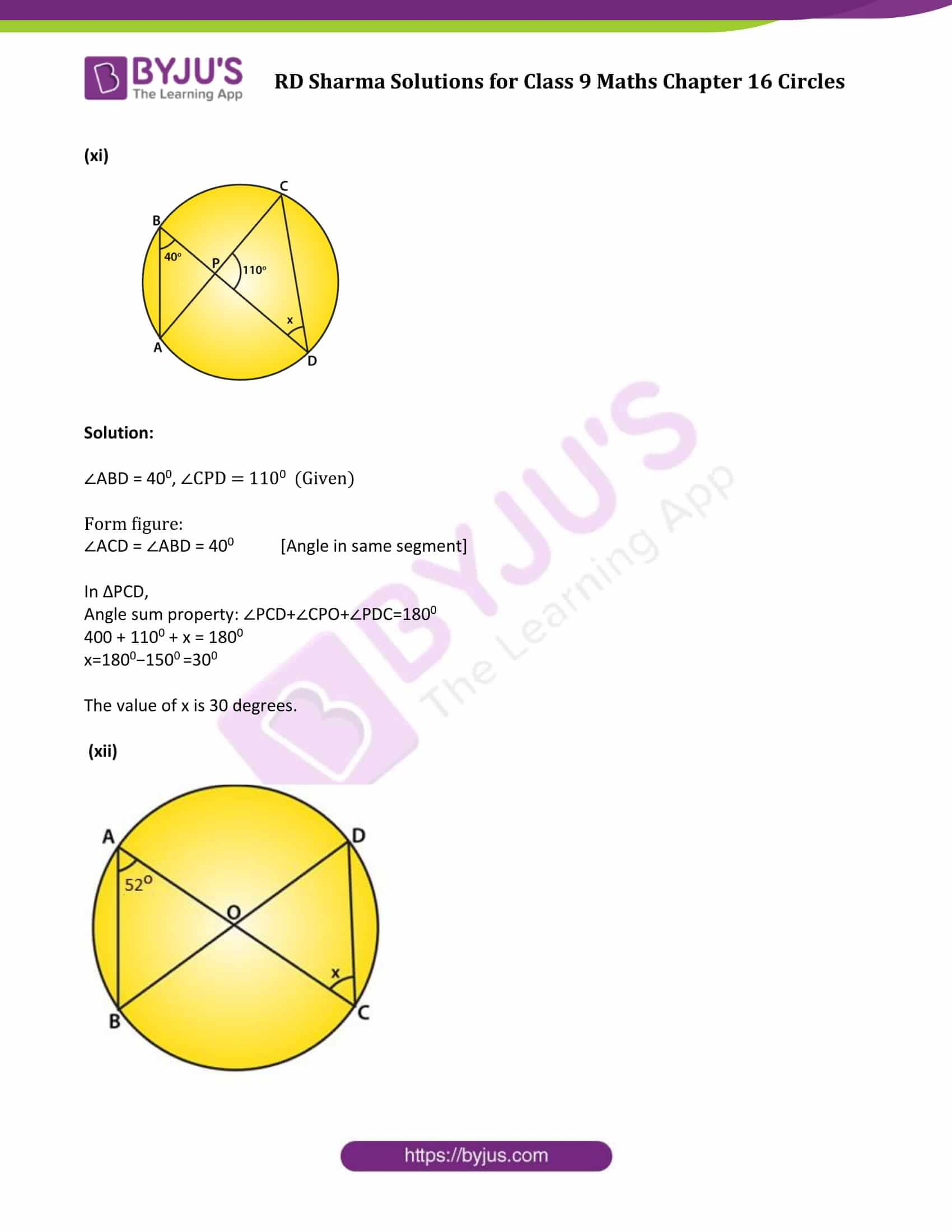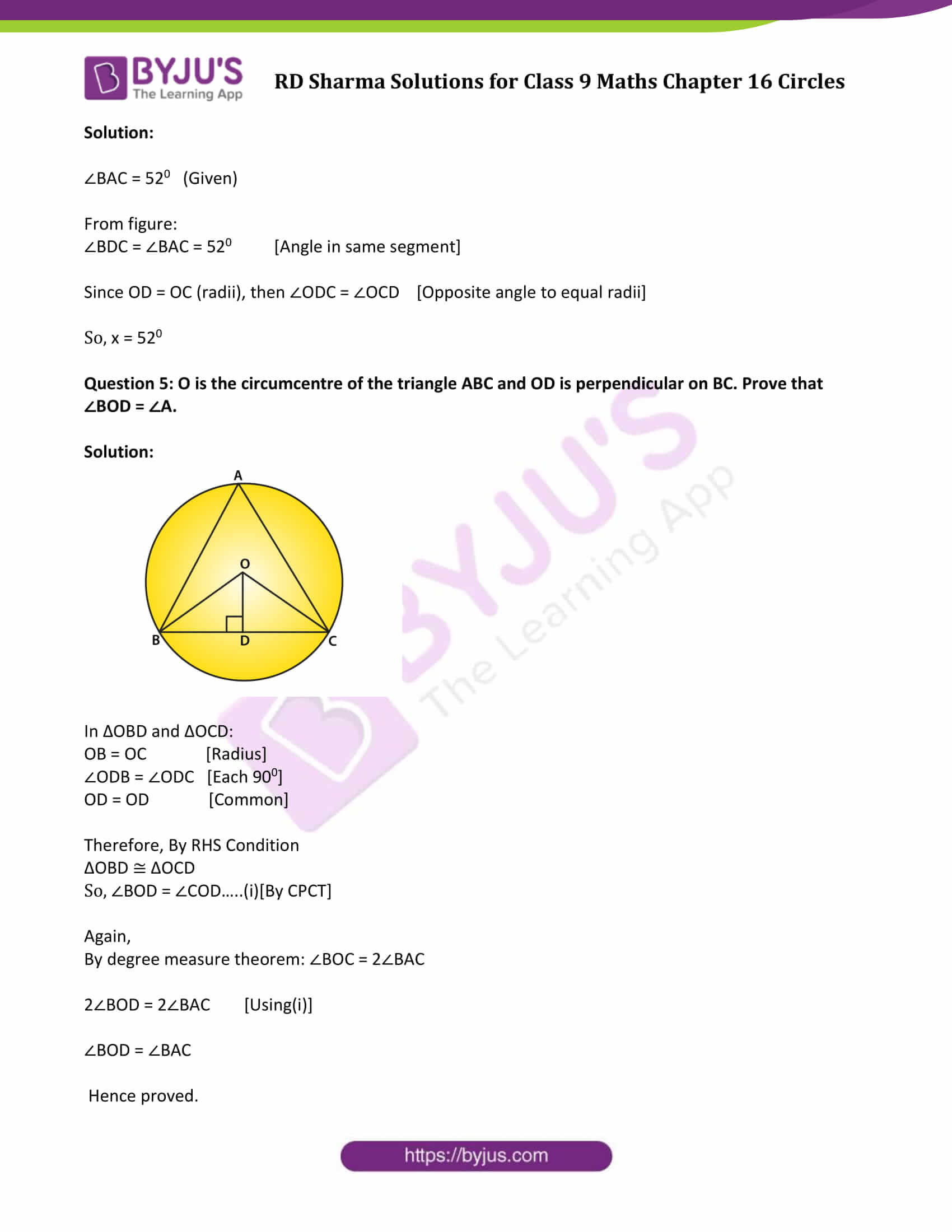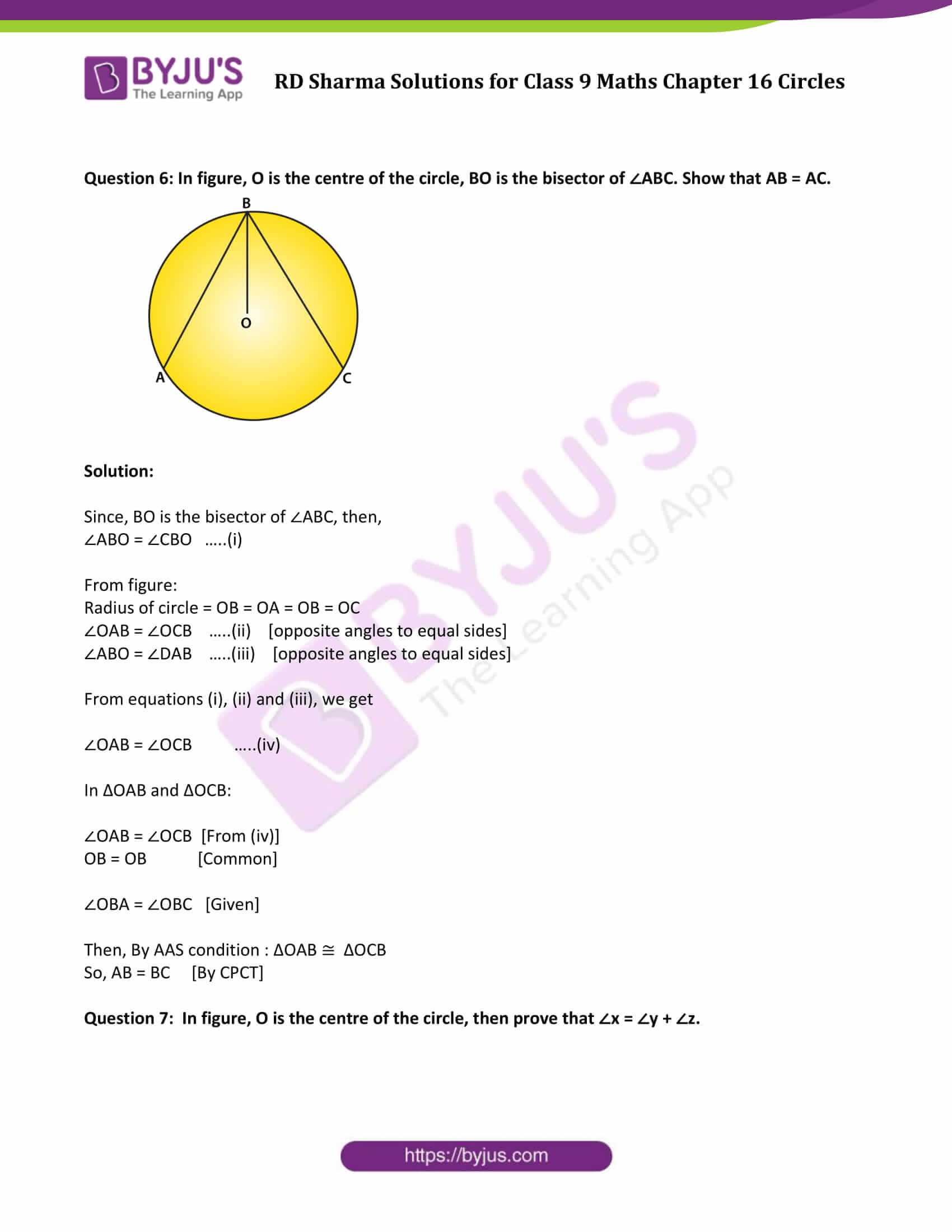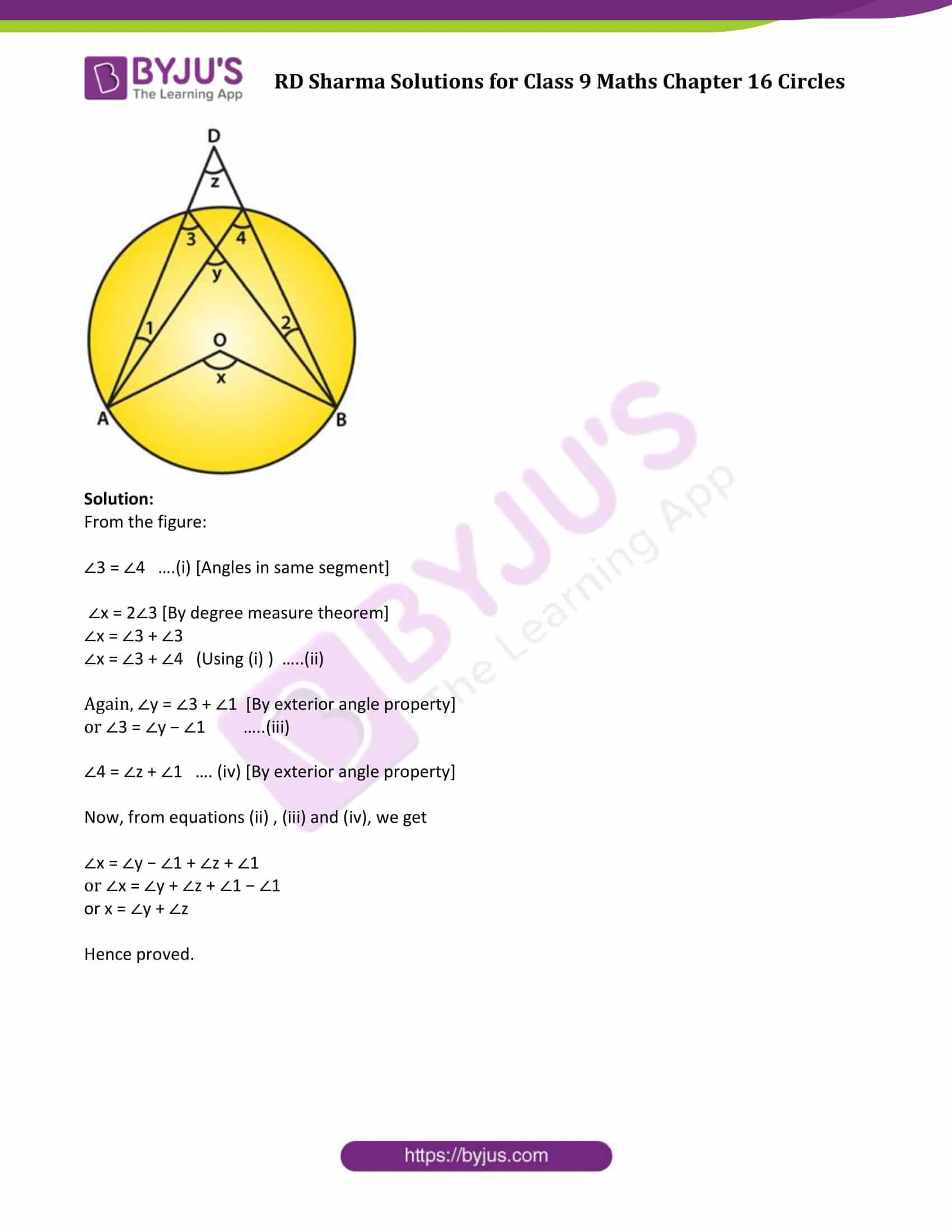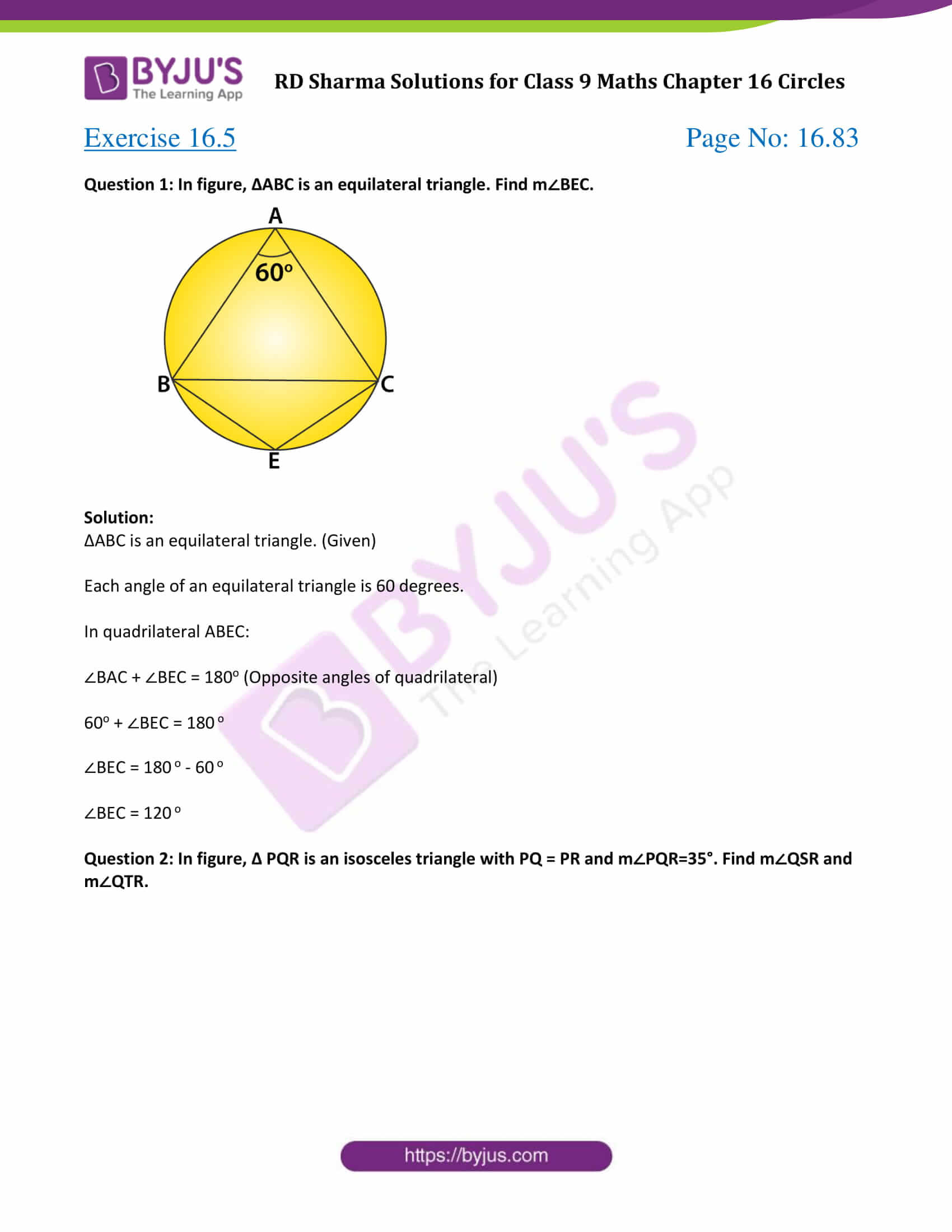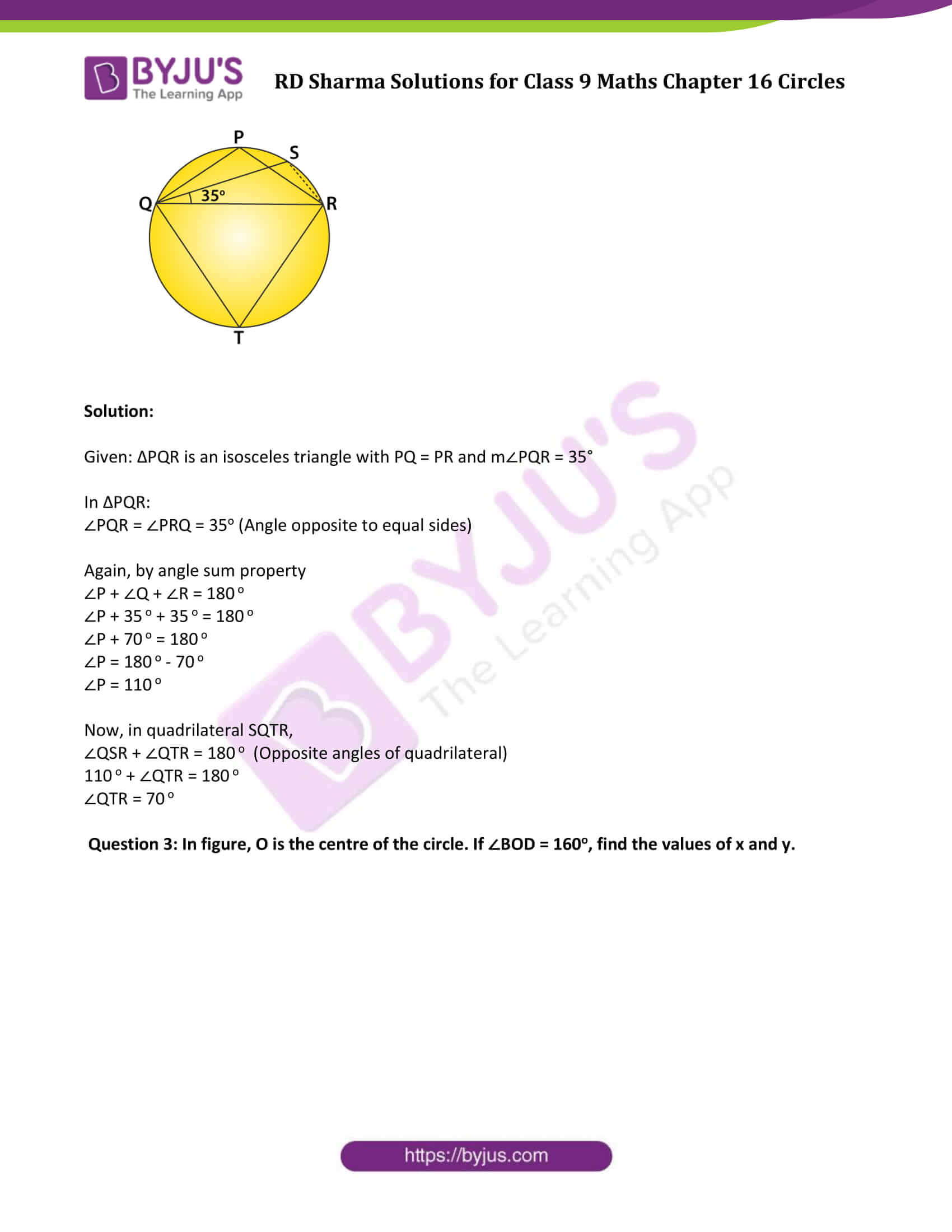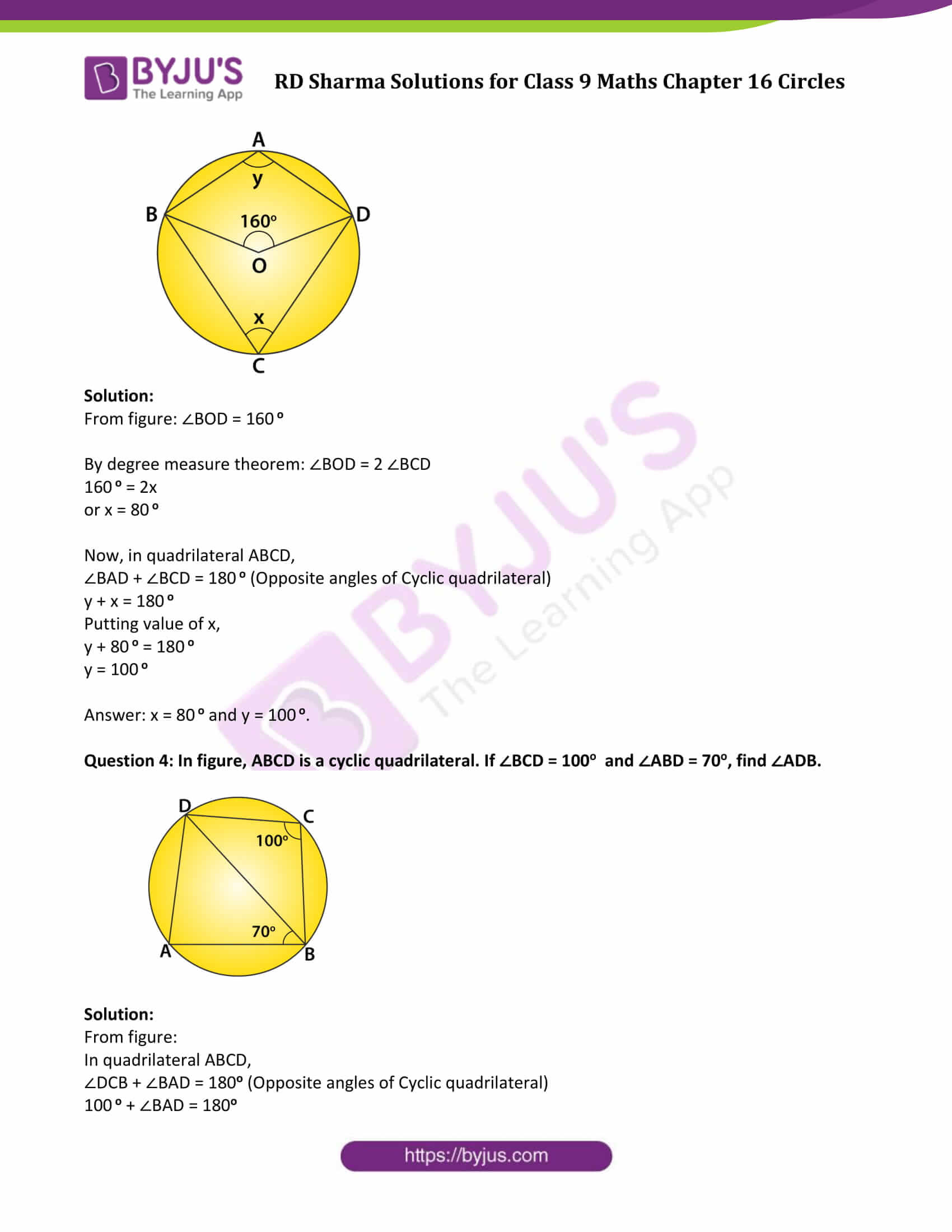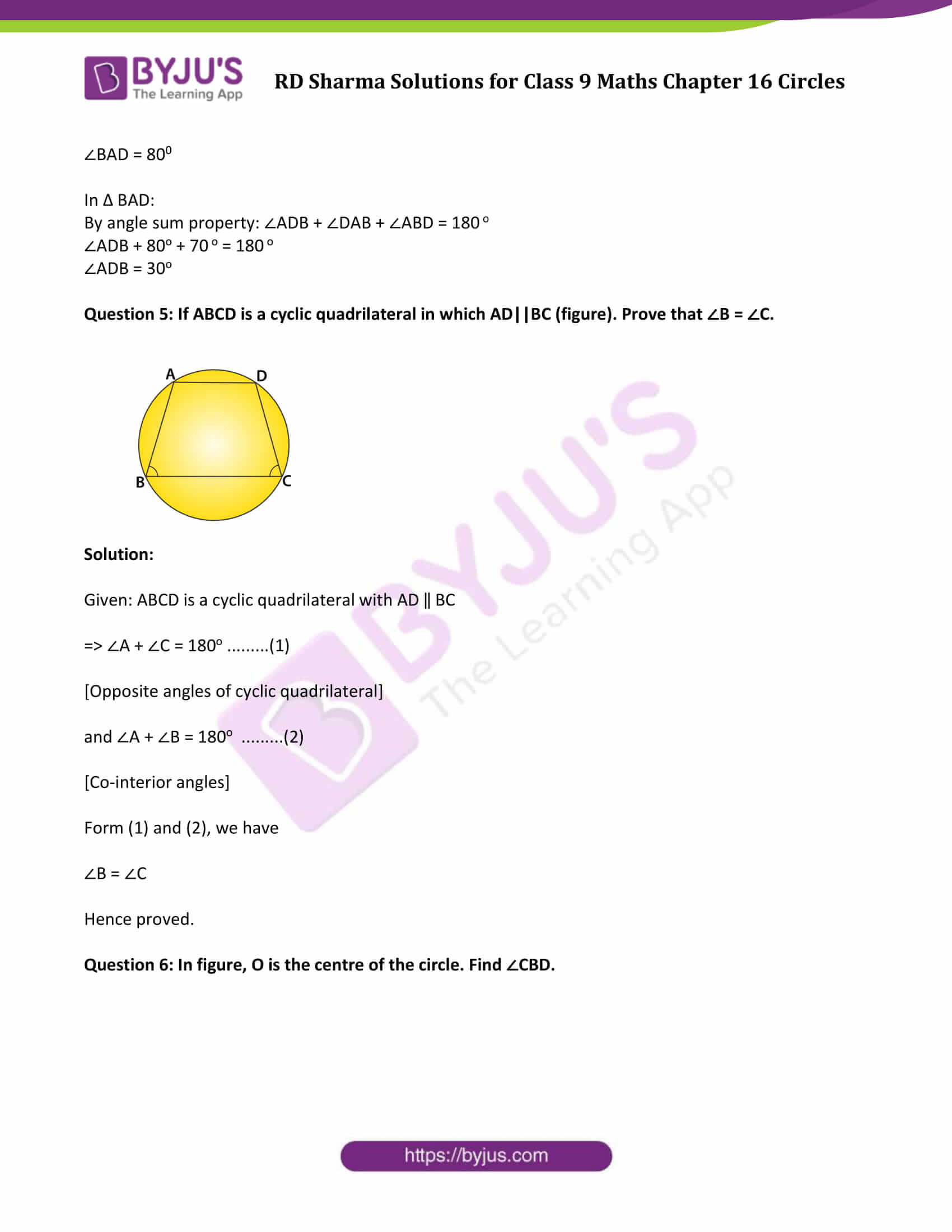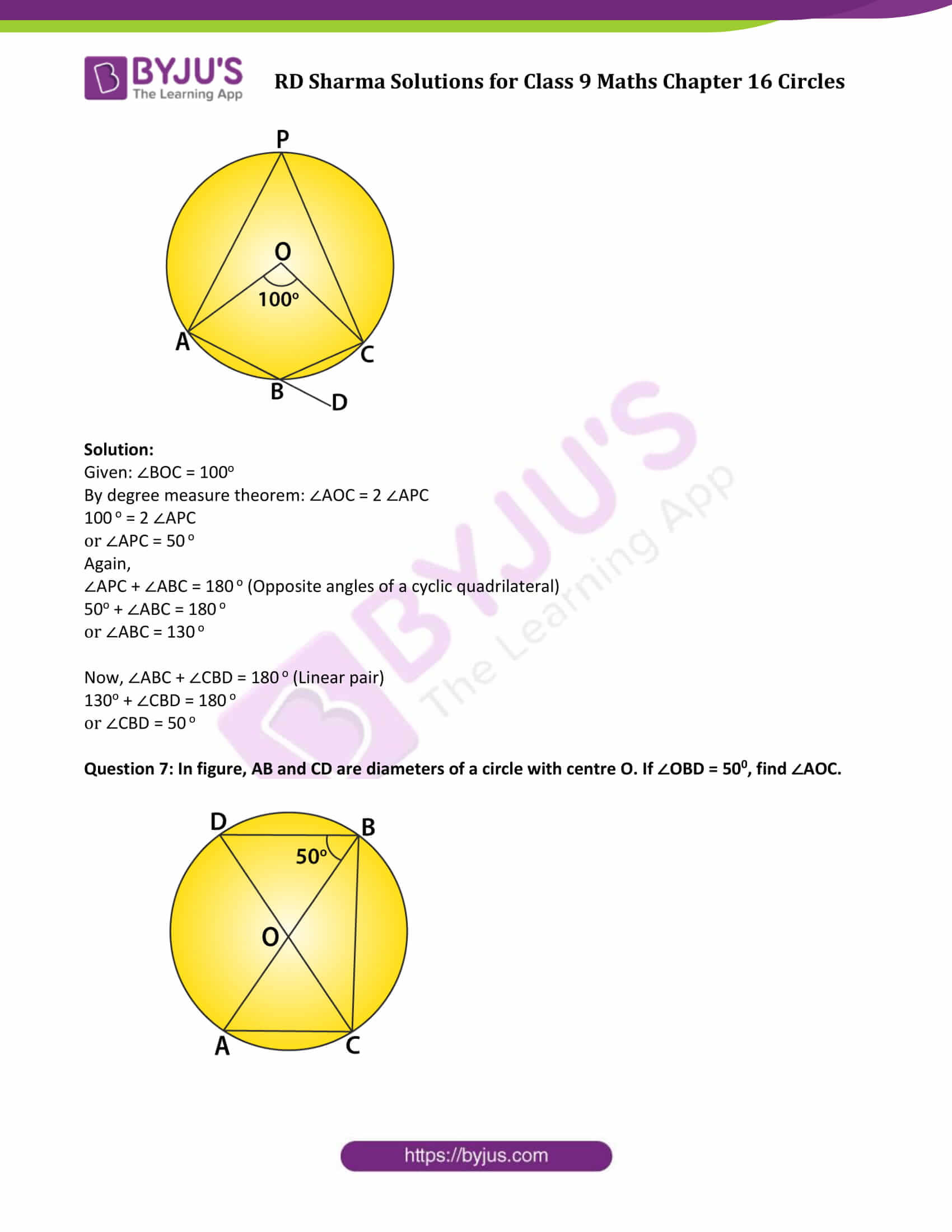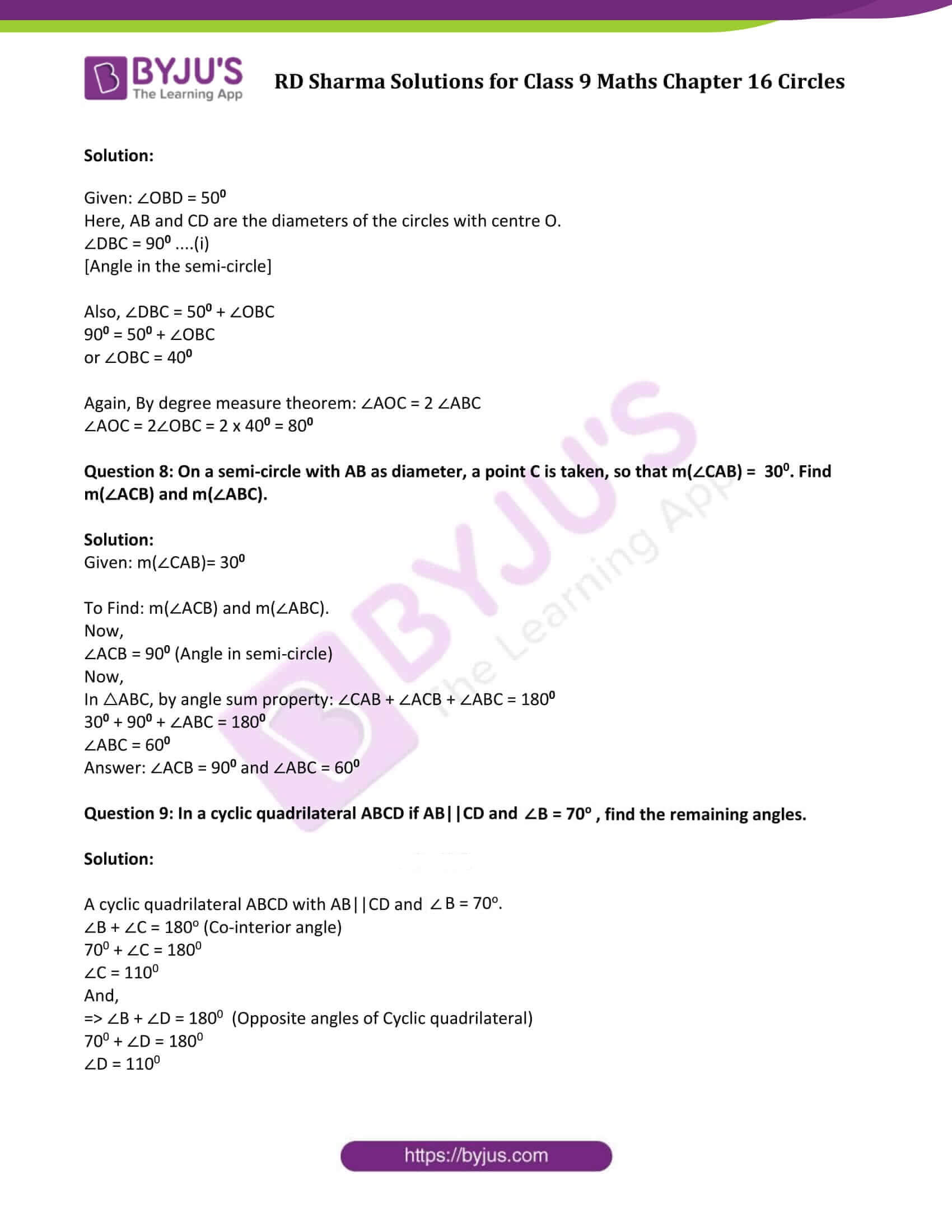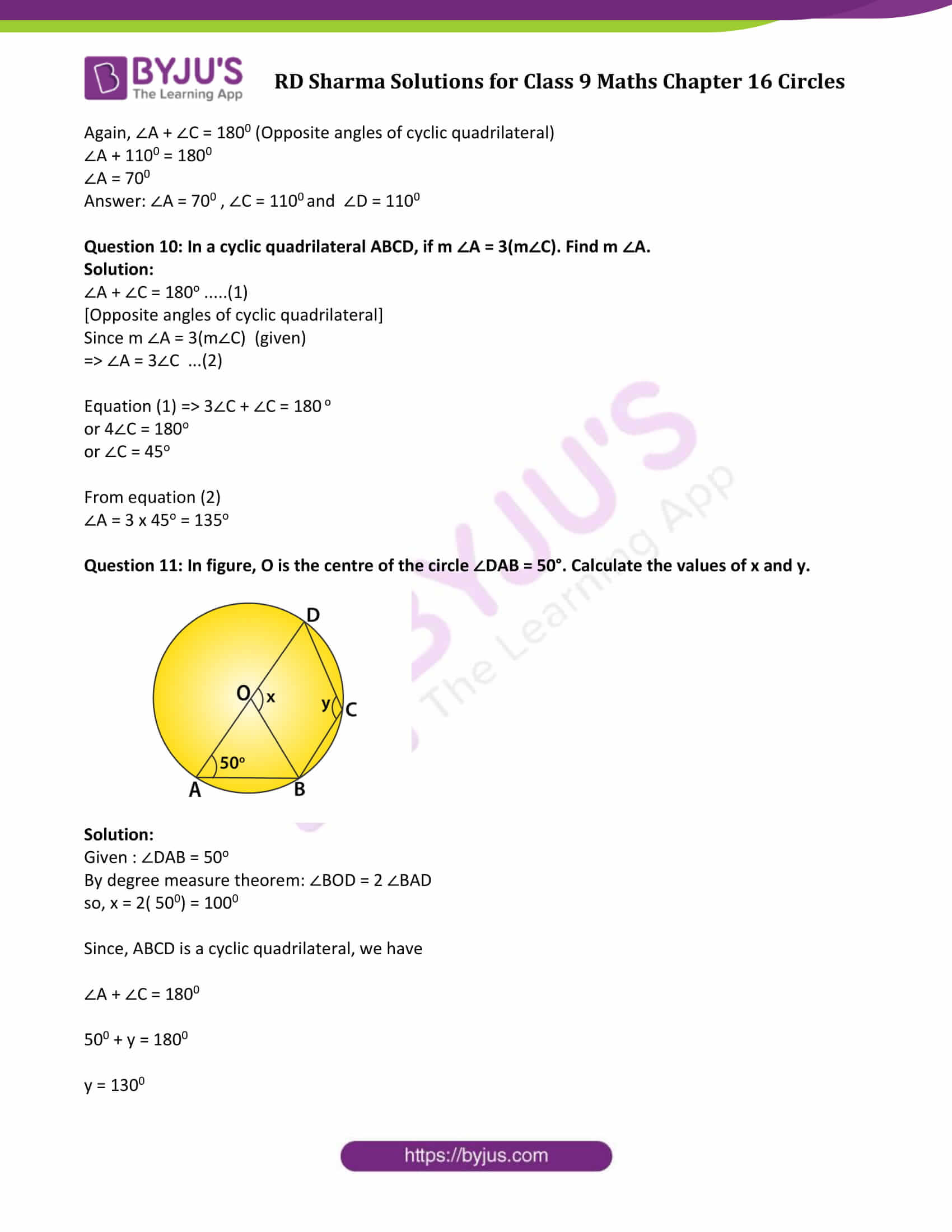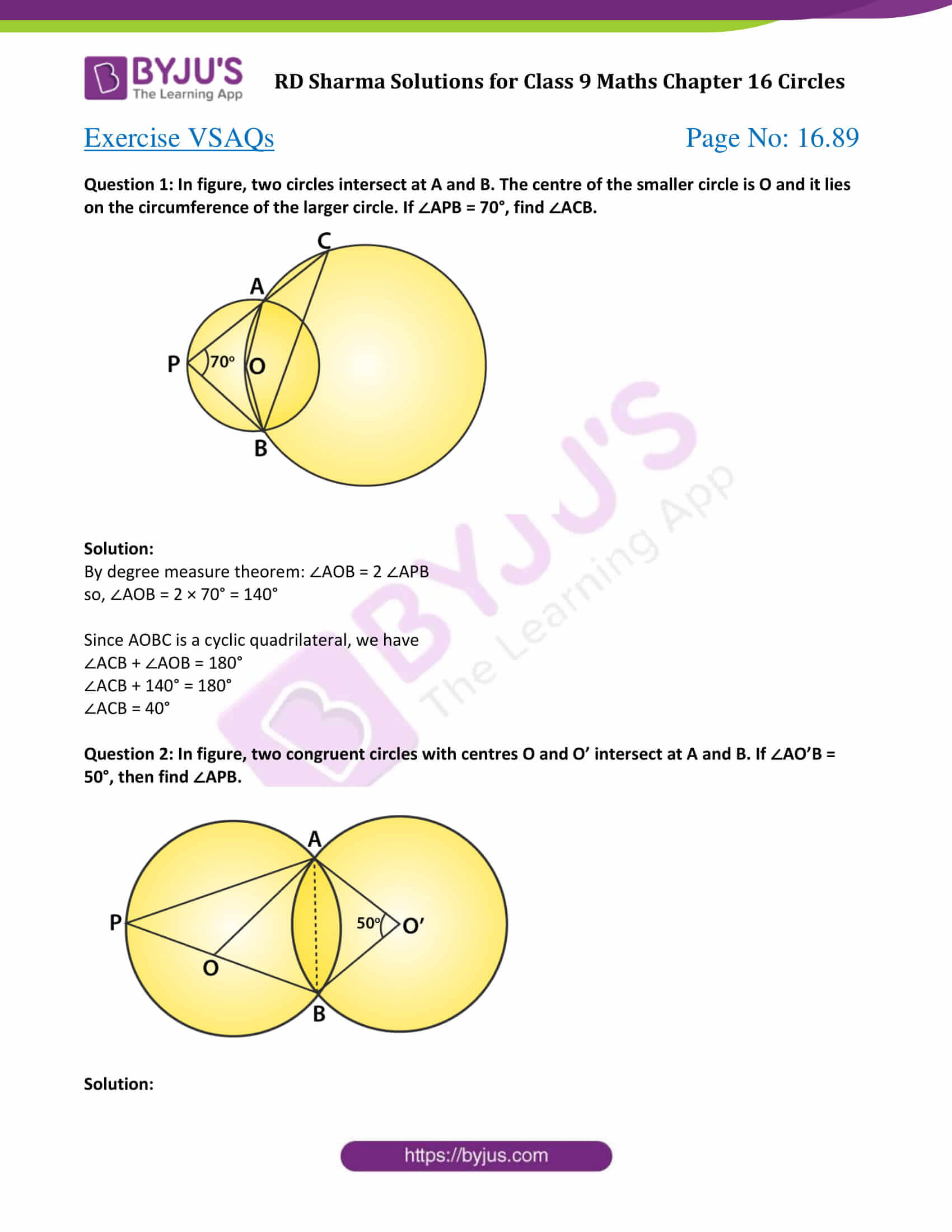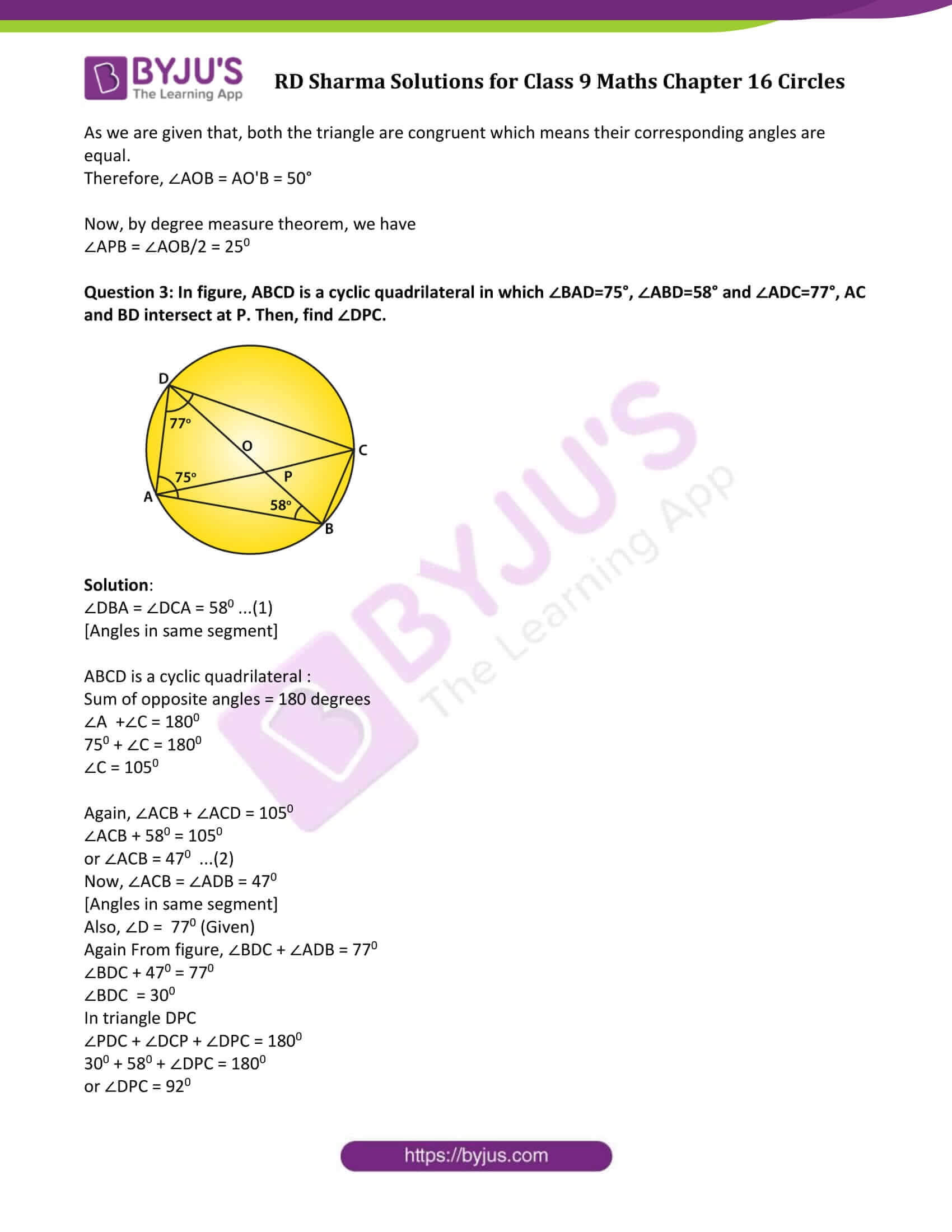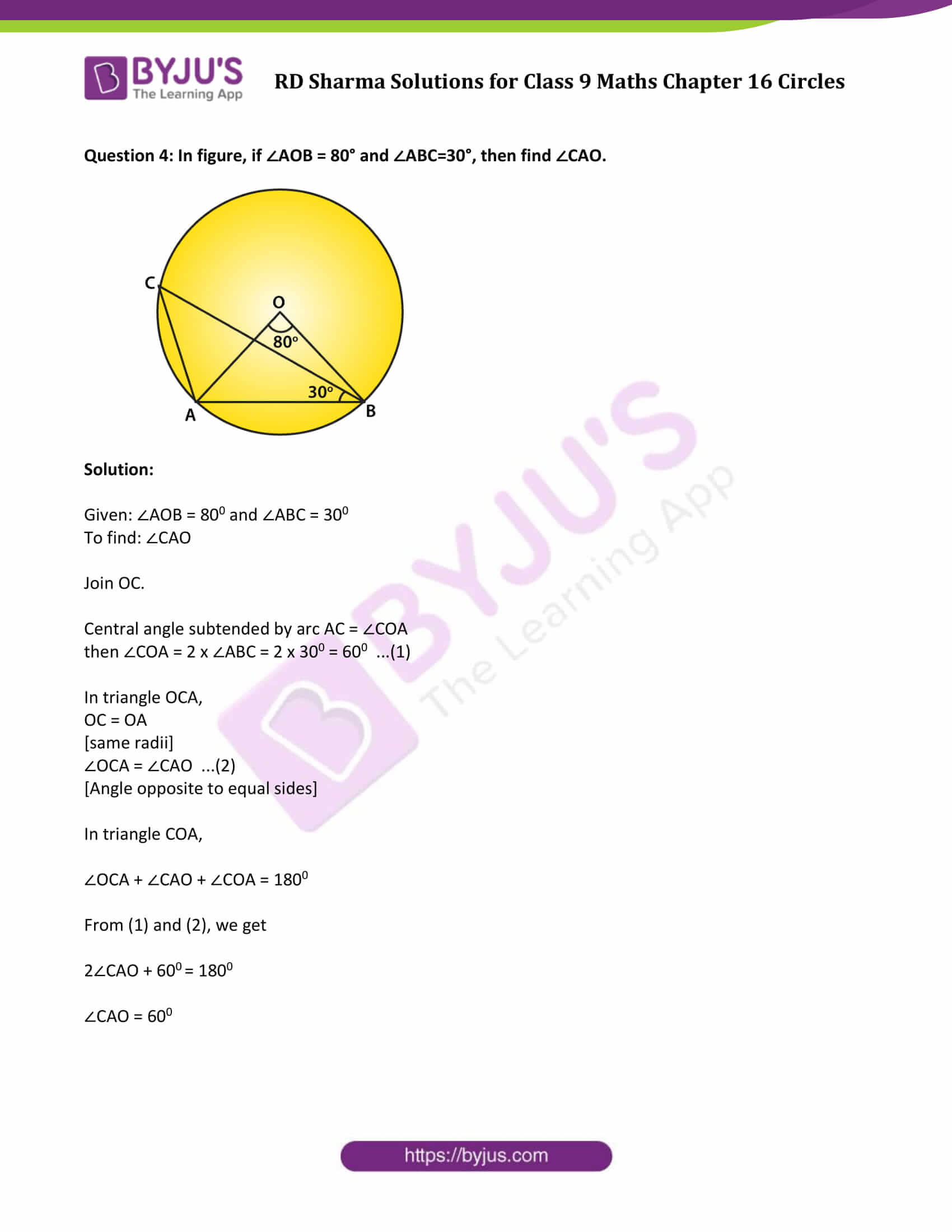### Exercise 16.1 Page No: 16.5

Question 1: Fill in the blanks:

(i) All points lying inside/outside a circle are called ______ points/_______ points.

(ii) Circles having the same centre and different radii are called _____ circles.

(iii) A point whose distance from the center of a circle is greater than its radius lies in _________ of the circle.

(iv) A continuous piece of a circle is _______ of the circle.

(v) The longest chord of a circle is a ____________ of the circle.

(vi) An arc is a __________ when its ends are the ends of a diameter.

(vii) Segment of a circle is a region between an arc and _______ of the circle.

(viii) A circle divides the plane, on which it lies, in _________ parts.

Solution:

(i) Interior/Exterior

(ii) Concentric

(iii) The Exterior

(iv) Arc

(v) Diameter

(vi) Semi-circle

(vii) Center

(viii) Three

Question 2: Write the truth value (T/F) of the following with suitable reasons:

(i) A circle is a plane figure.

(ii) Line segment joining the center to any point on the circle is a radius of the circle,

(iii) If a circle is divided into three equal arcs each is a major arc.

(iv) A circle has only finite number of equal chords.

(v) A chord of a circle, which is twice as long as its radius is the diameter of the circle.

(vi) Sector is the region between the chord and its corresponding arc.

(vii) The degree measure of an arc is the complement of the central angle containing the arc.

(viii) The degree measure of a semi-circle is 1800.

Solution:

(i) T

(ii) T

(iii) T

(iv) F

(v) T

(vi) T

(vii) F

(viii) T

### Exercise 16.2 Page No: 16.24

Question 1: The radius of a circle is 8 cm and the length of one of its chords is 12 cm. Find the distance of the chord from the centre.

Solution: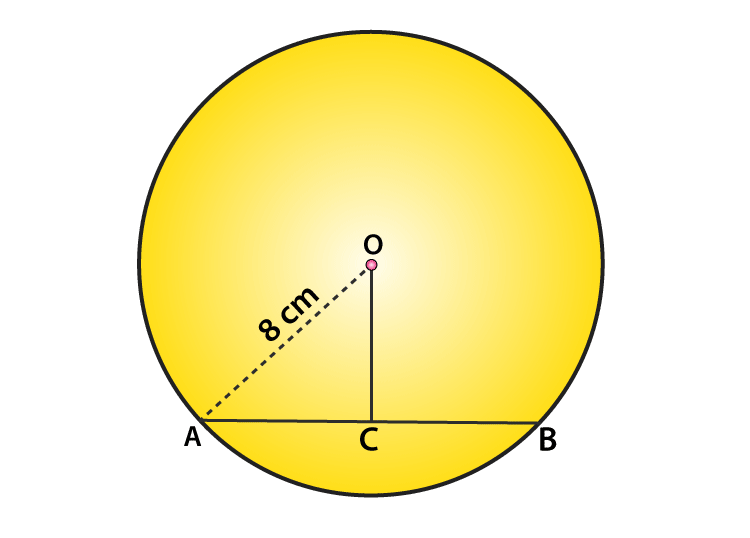Radius of circle (OA) = 8 cm (Given)

Chord (AB) = 12cm (Given)

Draw a perpendicular OC on AB.

We know, perpendicular from centre to chord bisects the chord

Which implies, AC = BC = 12/2 = 6 cm

In right ΔOCA:

Using Pythagoras theorem,

OA2 = AC2 + OC2

64 = 36 + OC2

OC2 = 64 – 36 = 28

or OC = √28 = 5.291 (approx.)

The distance of the chord from the centre is 5.291 cm.

Question 2: Find the length of a chord which is at a distance of 5 cm from the centre of a circle of radius 10 cm.

Solution: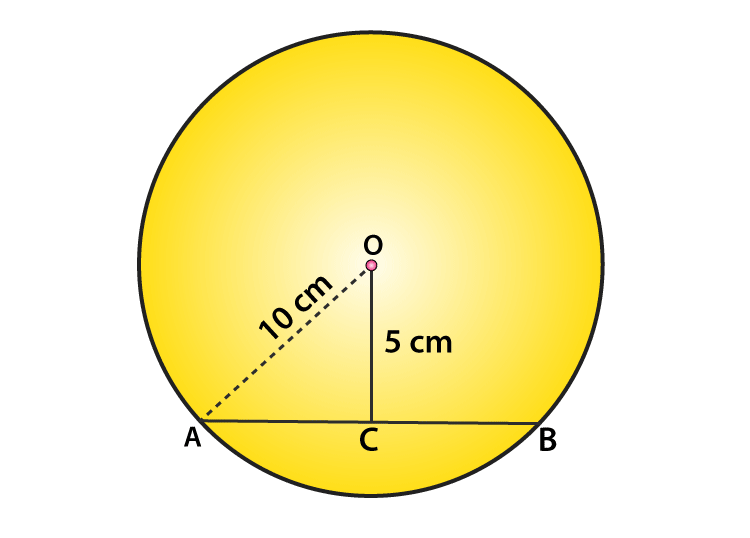Distance of the chord from the centre = OC = 5 cm (Given)

Radius of the circle = OA = 10 cm (Given)

In ΔOCA:

Using Pythagoras theorem,

OA2 = AC2 + OC2

100 = AC2 + 25

AC2 = 100 – 25 = 75

AC = √75 = 8.66

As, perpendicular from the centre to chord bisects the chord.

Therefore, AC = BC = 8.66 cm

=> AB = AC + BC = 8.66 + 8.66 = 17.32

Answer: AB = 17.32 cm

Question 3: Find the length of a chord which is at a distance of 4 cm from the centre of a circle of radius 6 cm.

Solution: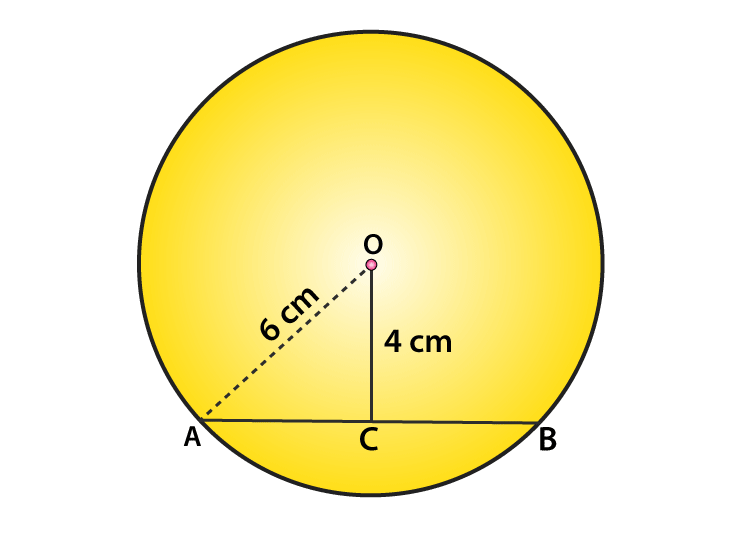Distance of the chord from the centre = OC = 4 cm (Given)

Radius of the circle = OA = 6 cm (Given)

In ΔOCA:

Using Pythagoras theorem,

OA2 = AC2 + OC2

36 = AC2 + 16

AC2 = 36 – 16 = 20

AC = √20 = 4.47

Or AC = 4.47cm

As, perpendicular from the centre to chord bisects the chord.

Therefore, AC = BC = 4.47 cm

=> AB = AC + BC = 4.47 + 4.47 = 8.94

Answer: AB = 8.94 cm

Question 4: Two chords AB, CD of lengths 5 cm, 11 cm respectively of a circle are parallel. If the distance between AB and CD is 3 cm, find the radius of the circle.

Solution: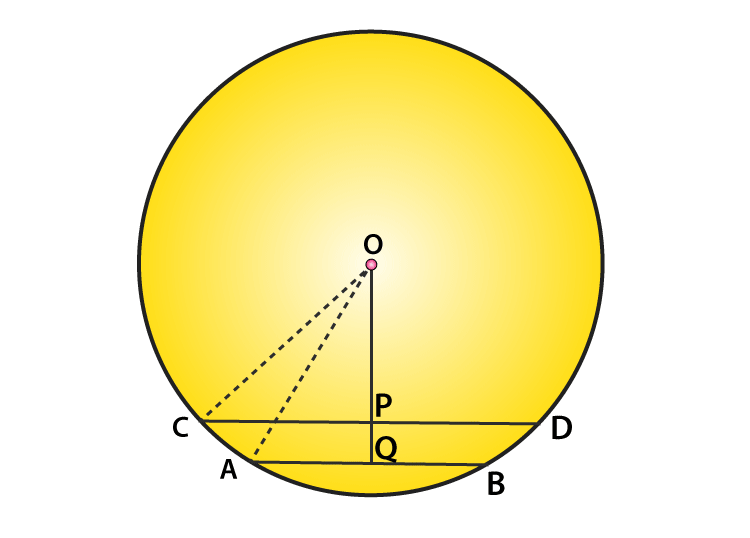Given: AB = 5 cm, CD = 11 cm, PQ = 3 cm

Draw perpendiculars OP on CD and OQ on AB

Let OP = x cm and OC = OA = r cm

We know, perpendicular from centre to chord bisects it.

Since OP⊥CD, we have

CP = PD = 11/2 cm

And OQ⊥AB

AQ = BQ = 5/2 cm

In ΔOCP:

By Pythagoras theorem,

OC2 = OP2 + CP2

r2 = x2 + (11/2) 2 …..(1)

In ΔOQA:

By Pythagoras theorem,

OA2=OQ2+AQ2

r2= (x+3) 2 + (5/2) 2 …..(2)

From equations (1) and (2), we get

(x+3) 2 + (5/2) 2 = x2 + (11/2) 2

Solve above equation and find the value of x.

x2 + 6x + 9 + 25/4 = x2 + 121/4

(using identity, (a+b) 2 = a2 + b2 + 2ab )

6x = 121/4 – 25/4 − 9

6x = 15

or x = 15/6 = 5/2

Substitute the value of x in equation (1), and find the length of radius,

r2 = (5/2)2 + (11/2) 2

= 25/4 + 121/4

= 146/4

or r = √146/4 cm

Question 5: Give a method to find the centre of a given circle.

Solution:

Steps of Construction:

Step 1: Consider three points A, B and C on a circle.

Step 2: Join AB and BC.

Step 3: Draw perpendicular bisectors of chord AB and BC which intersect each other at a point, say O.

Step 4: This point O is a centre of the circle, because we know that, the Perpendicular bisectors of chord always pass through the centre.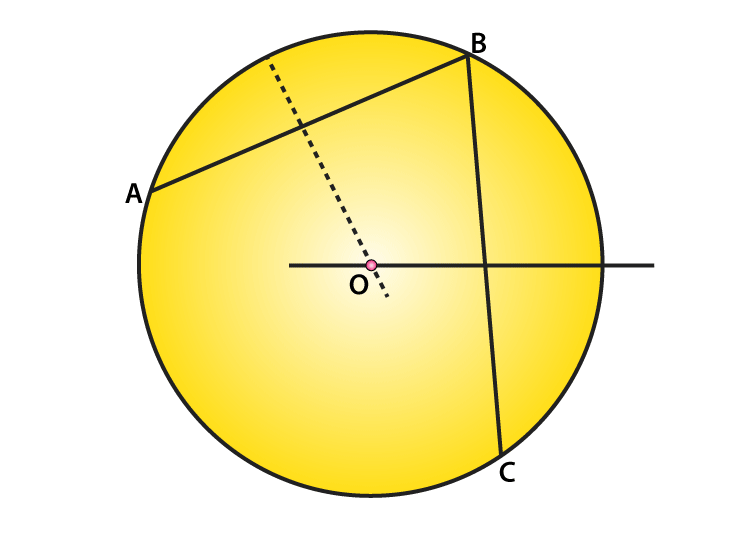Question 6: Prove that the line joining the mid-point of a chord to the centre of the circle passes through the mid-point of the corresponding minor arc.

Solution: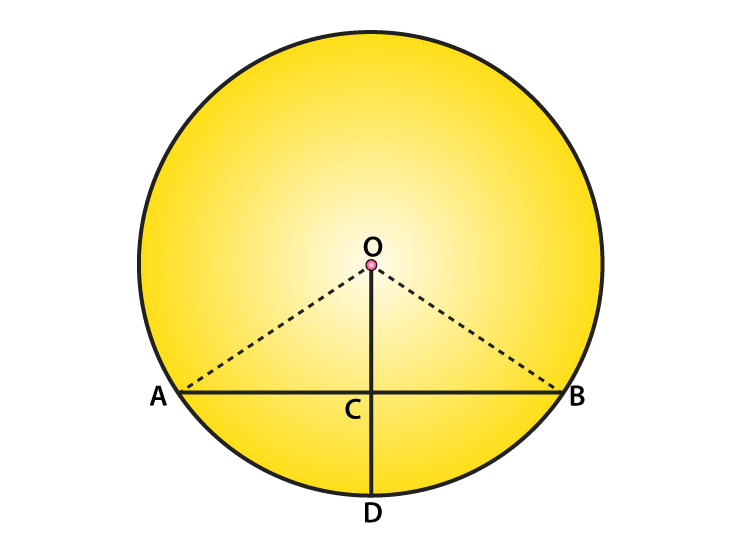From figure, Let C is the mid-point of chord AB.

To prove: D is the mid-point of arc AB.

Now, In ΔOAC and ΔOBC

OA = OB [Radius of circle]

OC = OC [Common]

AC = BC [C is the mid-point of chord AB (given)]

So, by SSS condition: ΔOAC ≅ ΔOBC

So, ∠AOC = ∠BOC (BY CPCT)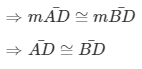Therefore, D is the mid-point of arc AB. Hence Proved.

Question 7: Prove that a diameter of a circle which bisects a chord of the circle also bisects the angle subtended by the chord at the centre of the circle.

Solution: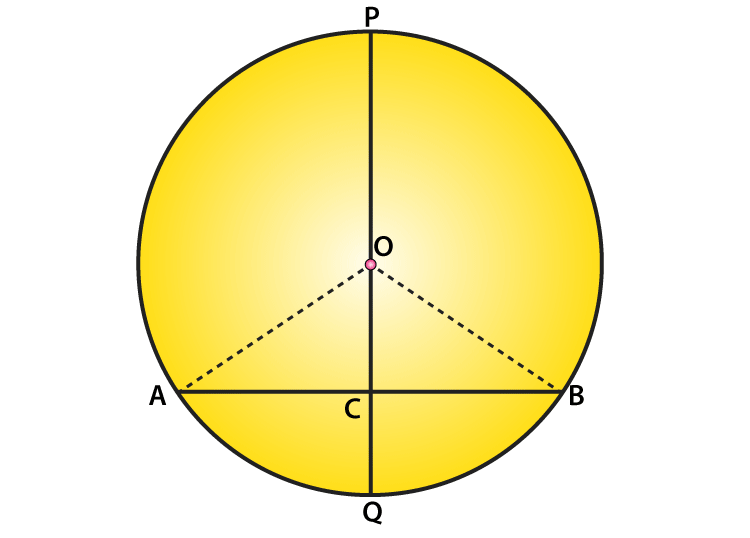Form figure: PQ is a diameter of circle which bisects the chord AB at C. (Given)

To Prove: PQ bisects ∠AOB

Now,

In ΔBOC and ΔAOC

OA = OB [Radius]

OC = OC [Common side]

AC = BC [Given]

Then, by SSS condition: ΔAOC ≅ ΔBOC

So, ∠AOC = ∠BOC [By c.p.c.t.]

Therefore, PQ bisects ∠AOB. Hence proved.

### Exercise 16.3 Page No: 16.40

Question 1: Three girls Ishita, Isha and Nisha are playing a game by standing on a circle of radius 20 m drawn in a park. Ishita throws a ball to Isha, Isha to Nisha and Nisha to Ishita. If the distance between Ishita and Isha and between Isha and Nisha is 24 m each, what is the distance between Ishita and Nisha.

Solution:

Let R, S and M be the position of Ishita, Isha and Nisha respectively.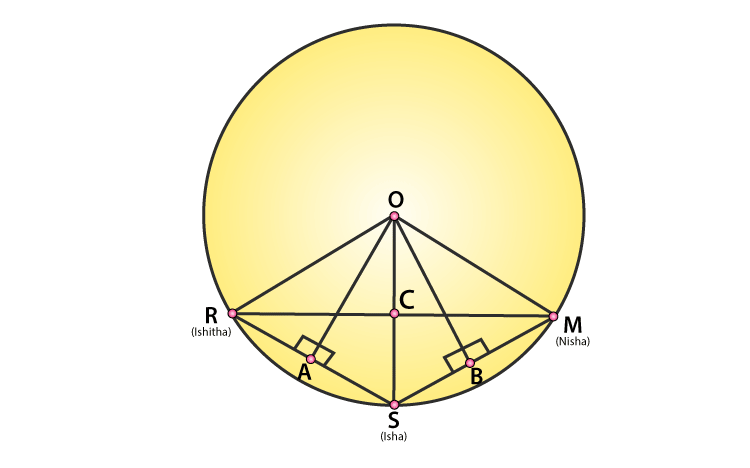Since OA is a perpendicular bisector on RS, so AR = AS = 24/2 = 12 cm

Radii of circle = OR = OS = OM = 20 cm (Given)

In ΔOAR:

By Pythagoras theorem,

OA2+AR2=OR2

OA2+122=202

OA2 = 400 – 144 = 256

Or OA = 16 m …(1)

From figure, OABC is a kite since OA = OC and AB = BC. We know that, diagonals of a kite are perpendicular and the diagonal common to both the isosceles triangles is bisected by another diagonal.

So in ΔRSM, ∠RCS = 900 and RC = CM …(2)

Now, Area of ΔORS = Area of ΔORS

=>1/2×OA×RS = 1/2 x RC x OS

=> OA ×RS = RC x OS

=> 16 x 24 = RC x 20

=> RC = 19.2

Since RC = CM (from (2), we have

RM = 2(19.2) = 38.4

So, the distance between Ishita and Nisha is 38.4 m.

Question 2: A circular park of radius 40 m is situated in a colony. Three boys Ankur, Amit and Anand are sitting at equal distance on its boundary each having a toy telephone in his hands to talk to each other. Find the length of the string of each phone.

Solution: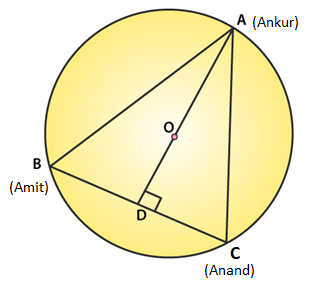Since, AB = BC = CA. So, ABC is an equilateral triangle

Radius = OA = 40 m (Given)

We know, medians of equilateral triangle pass through the circumcentre and intersect each other at the ratio 2 : 1.

Here AD is the median of equilateral triangle ABC, we can write:

OA/OD = 2/1

or 40/OD = 2/1

or OD = 20 m

Therefore, AD = OA + OD = (40 + 20) m = 60 m

By Pythagoras theorem,

AC2 = AD2 + DC2

AC2 = 602 + (AC/2) 2

AC2 = 3600 + AC2 / 4

3/4 AC2 = 3600

AC2 = 4800

or AC = 40√3 m

Therefore, length of string of each phone will be 40√3 m.

### Exercise 16.4 Page No: 16.60

Question 1: In figure, O is the centre of the circle. If ∠APB = 500, find ∠AOB and ∠OAB.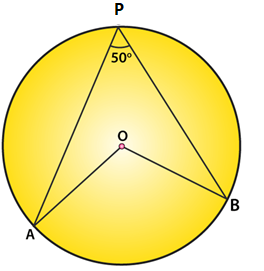Solution:

∠APB = 500 (Given)

By degree measure theorem: ∠AOB = 2∠APB

∠AOB = 2 × 500 = 1000

Again, OA = OB [Radius of circle]

Then ∠OAB = ∠OBA [Angles opposite to equal sides]

Let ∠OAB = m

In ΔOAB,

By angle sum property: ∠OAB+∠OBA+∠AOB=1800

=> m + m + 1000 = 1800

=>2m = 1800 – 1000 = 800

=>m = 800/2 = 400

∠OAB = ∠OBA = 400

Question 2: In figure, it is given that O is the centre of the circle and ∠AOC = 1500. Find ∠ABC.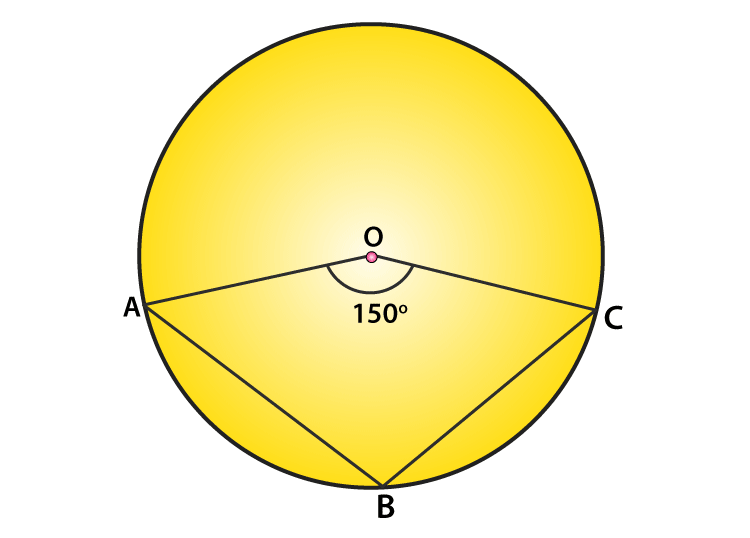Solution:

∠AOC = 1500 (Given)

By degree measure theorem: ∠ABC = (reflex∠AOC)/2 …(1)

We know, ∠AOC + reflex(∠AOC) = 3600 [Complex angle]

1500 + reflex∠AOC = 3600

or reflex ∠AOC = 3600−1500 = 2100

From (1) => ∠ABC = 210 o /2 = 105o

Question 3: In figure, O is the centre of the circle. Find ∠BAC.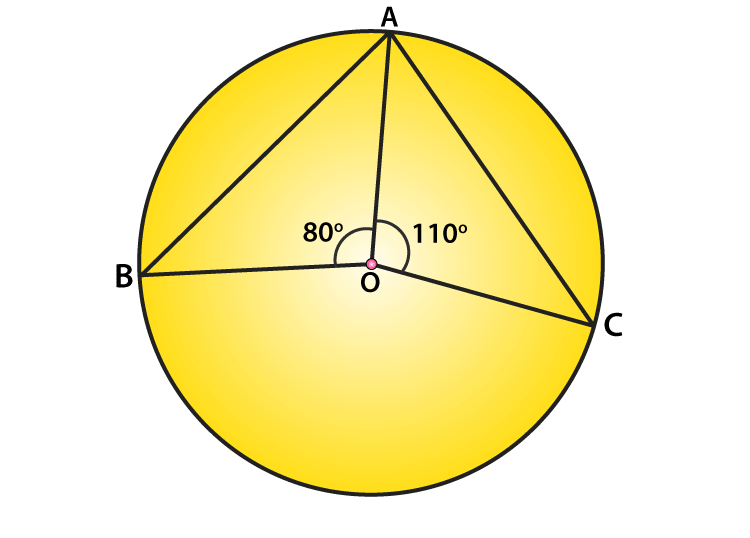Solution:

Given: ∠AOB = 800 and ∠AOC = 1100

Therefore, ∠AOB+∠AOC+∠BOC=3600 [Completeangle]

Substitute given values,

800 + 1000 + ∠BOC = 3600

∠BOC = 3600 – 800 – 1100 = 1700

or ∠BOC = 1700

Now, by degree measure theorem

∠BOC = 2∠BAC

1700 = 2∠BAC

Or ∠BAC = 1700/2 = 850

Question 4: If O is the centre of the circle, find the value of x in each of the following figures.

(i)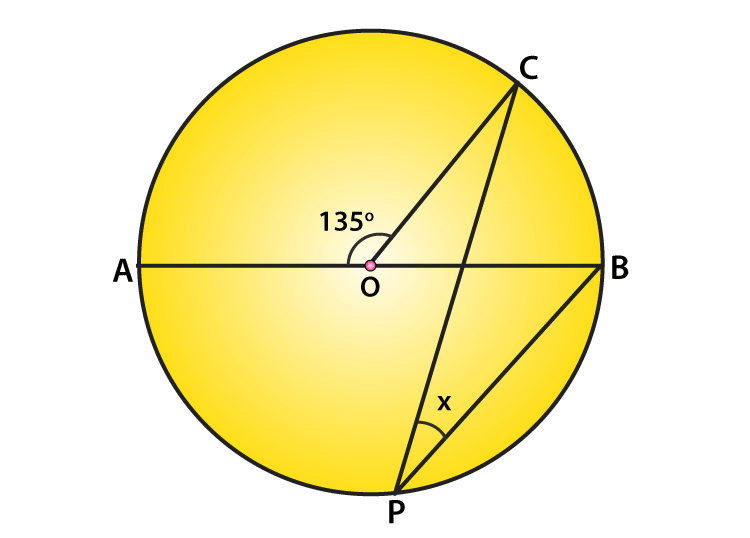Solution:

∠AOC = 1350 (Given)

From figure, ∠AOC + ∠BOC = 1800 [Linear pair of angles]

1350 +∠BOC = 1800

or ∠BOC=1800−1350

or ∠BOC=450

Again, by degree measure theorem

∠BOC = 2∠CPB

450 = 2x

x = 450/2

(ii)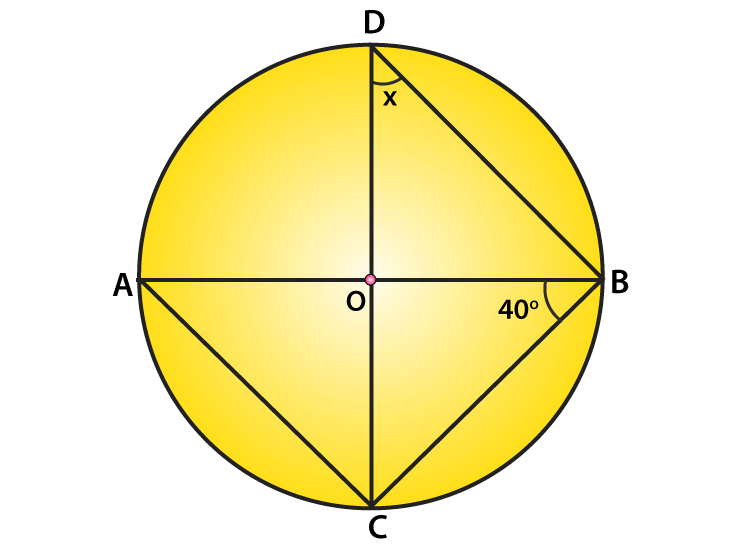Solution:

∠ABC=400 (given)

∠ACB = 900 [Angle in semicircle]

In ΔABC,

∠CAB+∠ACB+∠ABC=1800 [angle sum property]

∠CAB+900+400=1800

∠CAB=1800−900−400

∠CAB=500

Now, ∠CDB = ∠CAB [Angle is on same segment]

This implies, x = 500

(iii)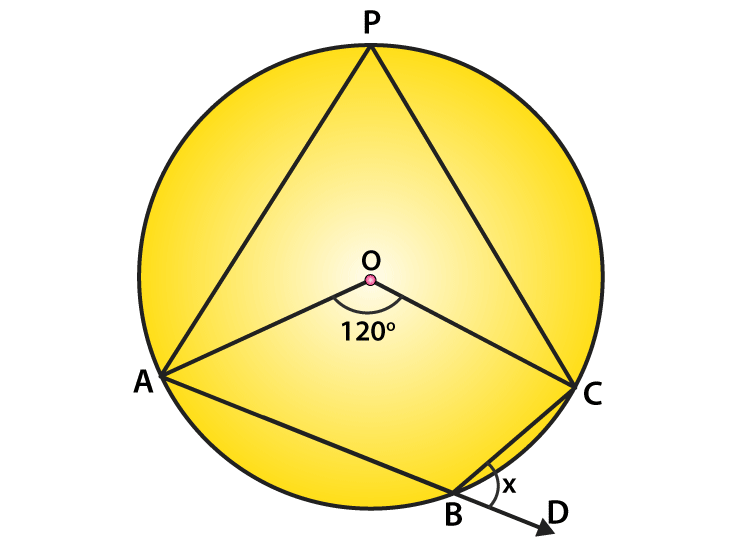Solution:

∠AOC = 1200 (given)

By degree measure theorem: ∠AOC = 2∠APC

1200 = 2∠APC

∠APC = 1200/2 = 600

Again, ∠APC + ∠ABC = 1800 [Sum of opposite angles of cyclic quadrilaterals = 180 o ]

600 + ∠ABC=1800

∠ABC=1800−600

∠ABC = 1200

∠ABC + ∠DBC = 1800 [Linear pair of angles]

1200 + x = 1800

x = 1800−1200=600

The value of x is 600

(iv)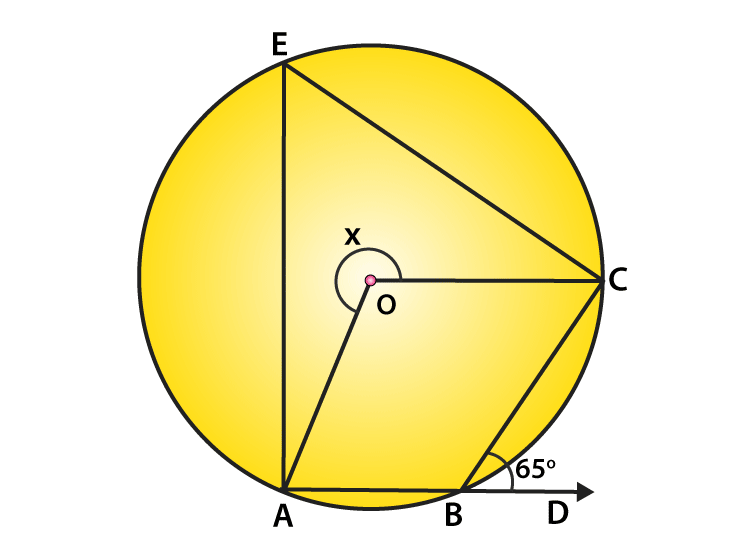Solution:

∠CBD = 650 (given)

From figure:

∠ABC + ∠CBD = 1800 [ Linear pair of angles]

∠ABC + 650 = 1800

∠ABC =1800−650=1150

Again, reflex ∠AOC = 2∠ABC [Degree measure theorem]

x=2(1150) = 2300

The value of x is 2300

(v)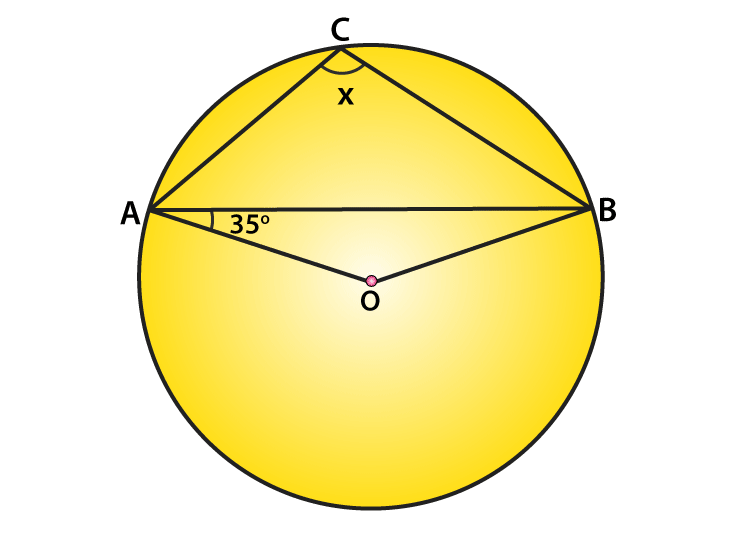Solution:

∠OAB = 350 (Given)

From figure:

∠OBA = ∠OAB = 350 [Angles opposite to equal radii]

InΔAOB:

∠AOB + ∠OAB + ∠OBA = 1800 [angle sum property]

∠AOB + 350 + 350 = 1800

∠AOB = 1800 – 350 – 350 = 1100

Now, ∠AOB + reflex∠AOB = 3600 [Complex angle]

1100 + reflex∠AOB = 3600

reflex∠AOB = 3600 – 1100 = 2500

By degree measure theorem: reflex ∠AOB = 2∠ACB

2500 = 2x

x = 2500/2=1250

(vi)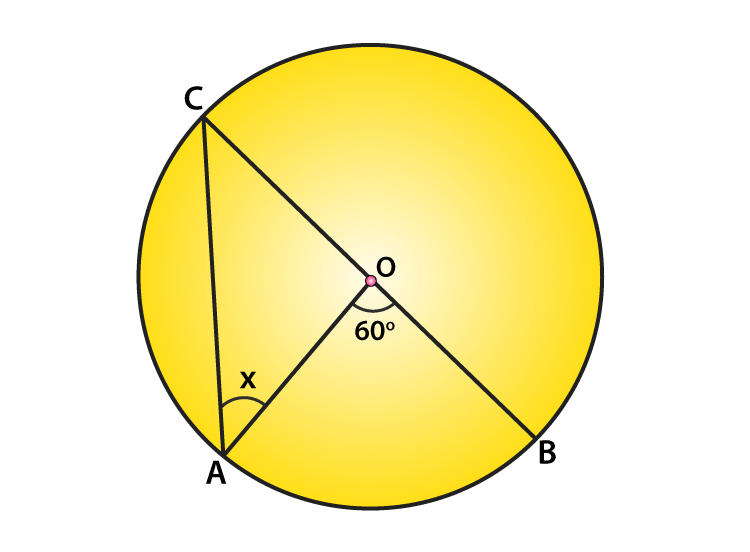Solution:

∠AOB = 60o (given)

By degree measure theorem: reflex∠AOB = 2∠OAC

60 o = 2∠ OAC

∠OAC = 60 o / 2 = 30 o [Angles opposite to equal radii]

Or x = 300

(vii)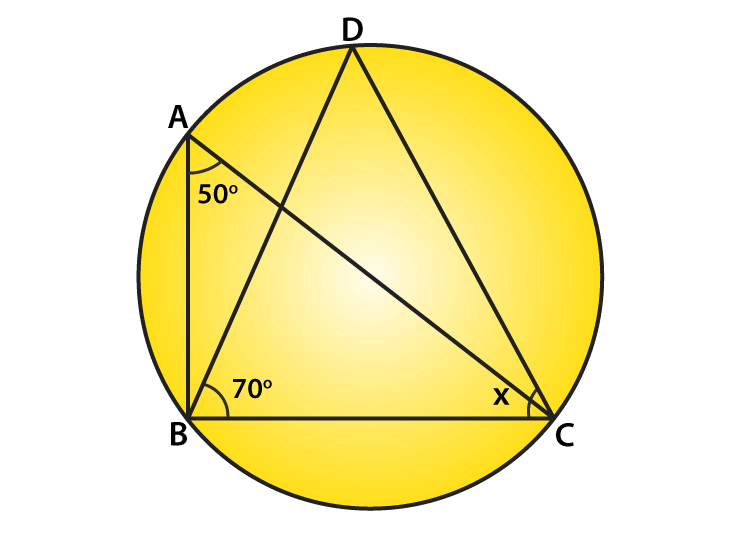Solution:

∠BAC = 500 and ∠DBC = 700 (given)

From figure:

∠BDC = ∠BAC = 500 [Angle on same segment]

Now,

In ΔBDC:

Using angle sum property, we have

∠BDC+∠BCD+∠DBC=1800

Substituting given values, we get

500 + x0 + 700 = 1800

x0 = 1800−500−700=600

or x = 60o

(viii)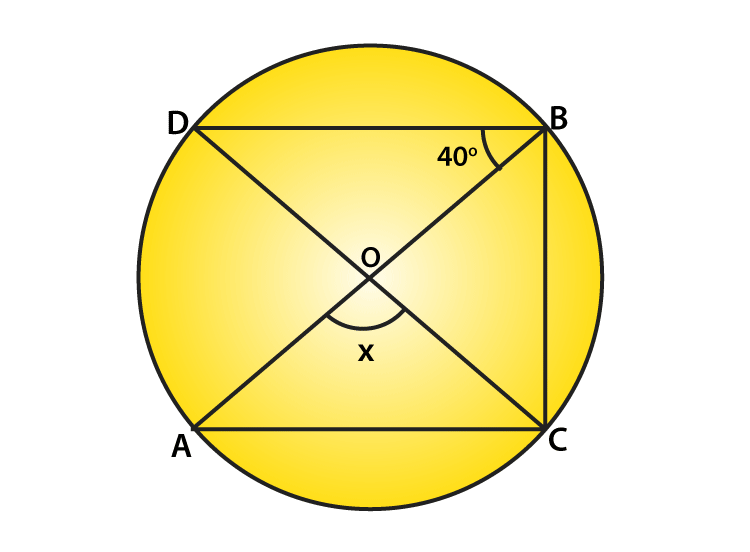Solution:

∠DBO = 400 (Given)

Form figure:

∠DBC = 900 [Angle in a semicircle]

∠DBO + ∠OBC = 900

400+∠OBC=900

or ∠OBC=900−400=500

Again, By degree measure theorem: ∠AOC = 2∠OBC

or x = 2×500=1000

(ix)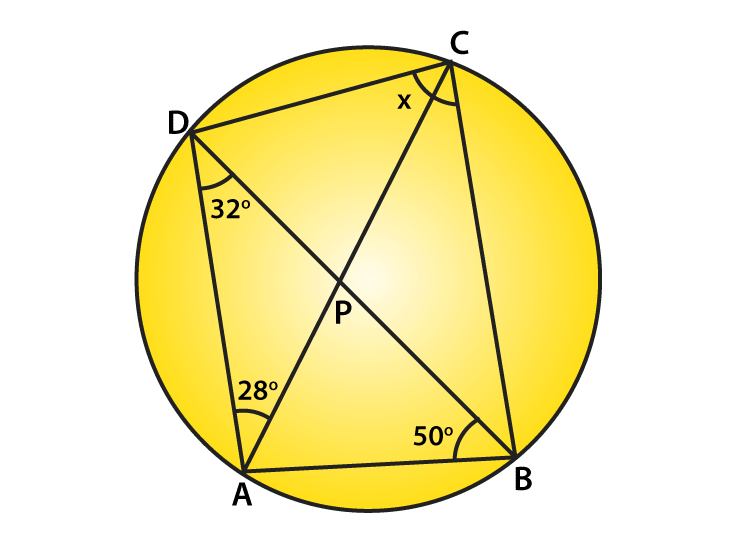Solution:

∠CAD = 28, ∠ADB = 32 and ∠ABC = 50 (Given)

From figure:

In ΔDAB:

Angle sum property: ∠ADB + ∠DAB + ∠ABD = 1800

By substituting the given values, we get

320 + ∠DAB + 500 = 1800

∠DAB=1800−320−500

∠DAB = 980

Now,

∠DAB+∠DCB=1800 [Opposite angles of cyclic quadrilateral, their sum = 180 degrees]

980+x=1800

or x = 1800−980=820

The value of x is 82 degrees.

(x)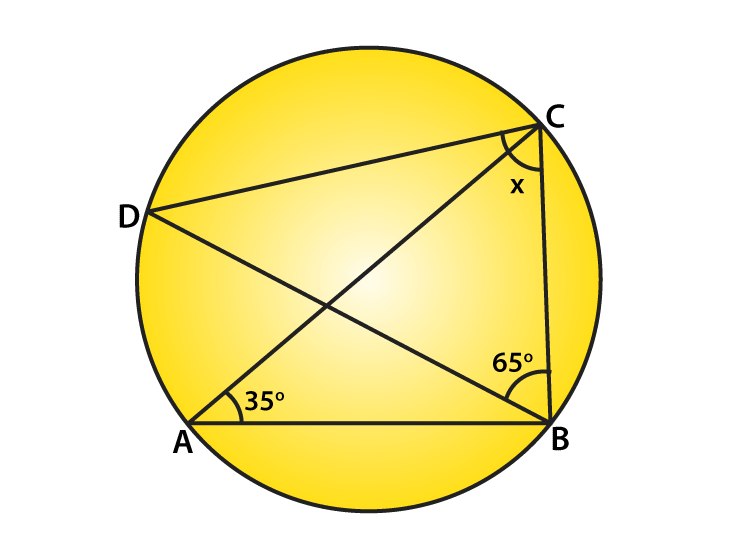Solution:

∠BAC = 350 and ∠DBC = 650

From figure:

∠BDC = ∠BAC = 350 [Angle in same segment]

In ΔBCD:

Angle sum property, we have

∠BDC + ∠BCD + ∠DBC = 1800

350 + x + 650 = 1800

or x = 1800 – 350 – 650 = 800

(xi)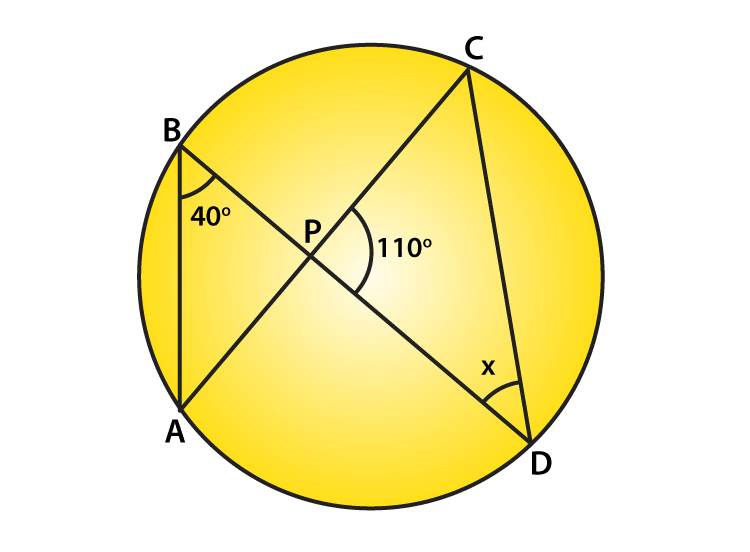Solution:

∠ABD = 400, ∠CPD = 1100 (Given)

Form figure:

∠ACD = ∠ABD = 400 [Angle in same segment]

In ΔPCD,

Angle sum property: ∠PCD+∠CPO+∠PDC=1800

400 + 1100 + x = 1800

x=1800−1500 =300

The value of x is 30 degrees.

(xii)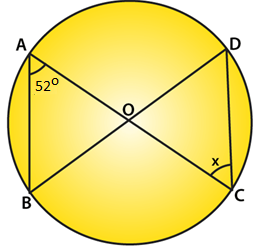Solution:

∠BAC = 520 (Given)

From figure:

∠BDC = ∠BAC = 520 [Angle in same segment]

Since OD = OC (radii), then ∠ODC = ∠OCD [Opposite angle to equal radii]

So, x = 520

Question 5: O is the circumcentre of the triangle ABC and OD is perpendicular on BC. Prove that ∠BOD = ∠A.

Solution: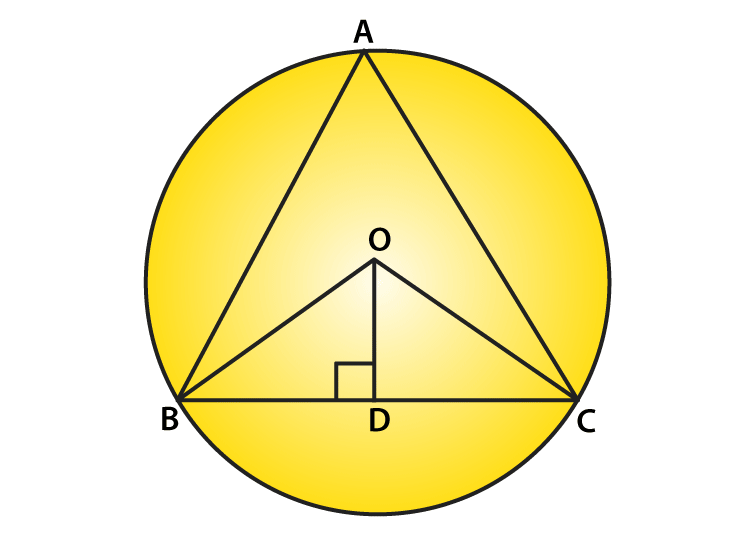In ΔOBD and ΔOCD:

OB = OC [Radius]

∠ODB = ∠ODC [Each 900]

OD = OD [Common]

Therefore, By RHS Condition

ΔOBD ≅ ΔOCD

So, ∠BOD = ∠COD…..(i)[By CPCT]

Again,

By degree measure theorem: ∠BOC = 2∠BAC

2∠BOD = 2∠BAC [Using(i)]

∠BOD = ∠BAC

Hence proved.

Question 6: In figure, O is the centre of the circle, BO is the bisector of ∠ABC. Show that AB = AC.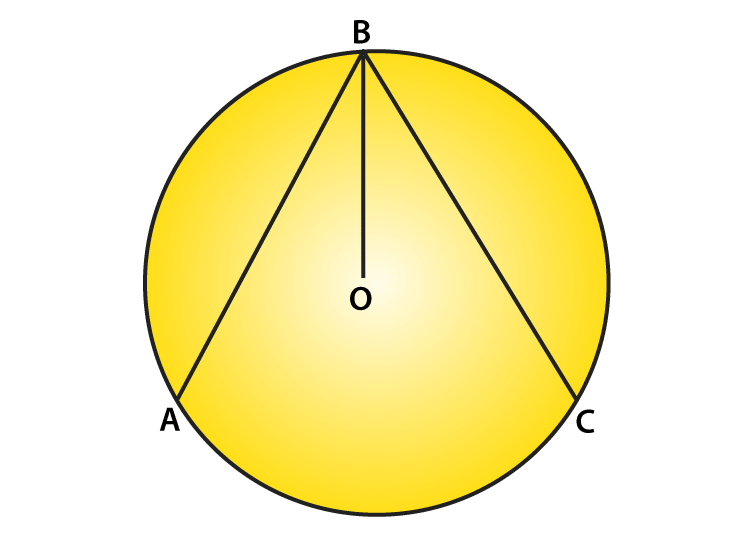Solution:

Since, BO is the bisector of ∠ABC, then,

∠ABO = ∠CBO …..(i)

From figure:

Radius of circle = OB = OA = OB = OC

∠OAB = ∠OCB …..(ii) [opposite angles to equal sides]

∠ABO = ∠DAB …..(iii) [opposite angles to equal sides]

From equations (i), (ii) and (iii), we get

∠OAB = ∠OCB …..(iv)

In ΔOAB and ΔOCB:

∠OAB = ∠OCB [From (iv)]

OB = OB [Common]

∠OBA = ∠OBC [Given]

Then, By AAS condition : ΔOAB ≅ ΔOCB

So, AB = BC [By CPCT]

Question 7: In figure, O is the centre of the circle, then prove that ∠x = ∠y + ∠z.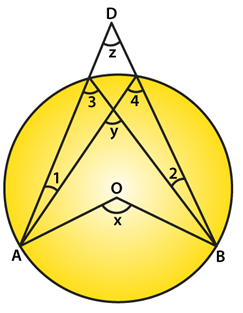Solution:

From the figure:

∠3 = ∠4 ….(i) [Angles in same segment]

∠x = 2∠3 [By degree measure theorem]

∠x = ∠3 + ∠3

∠x = ∠3 + ∠4 (Using (i) ) …..(ii)

Again, ∠y = ∠3 + ∠1 [By exterior angle property]

or ∠3 = ∠y − ∠1 …..(iii)

∠4 = ∠z + ∠1 …. (iv) [By exterior angle property]

Now, from equations (ii) , (iii) and (iv), we get

∠x = ∠y − ∠1 + ∠z + ∠1

or ∠x = ∠y + ∠z + ∠1 − ∠1

or x = ∠y + ∠z

Hence proved.

### Exercise 16.5 Page No: 16.83

Question 1: In figure, ΔABC is an equilateral triangle. Find m∠BEC.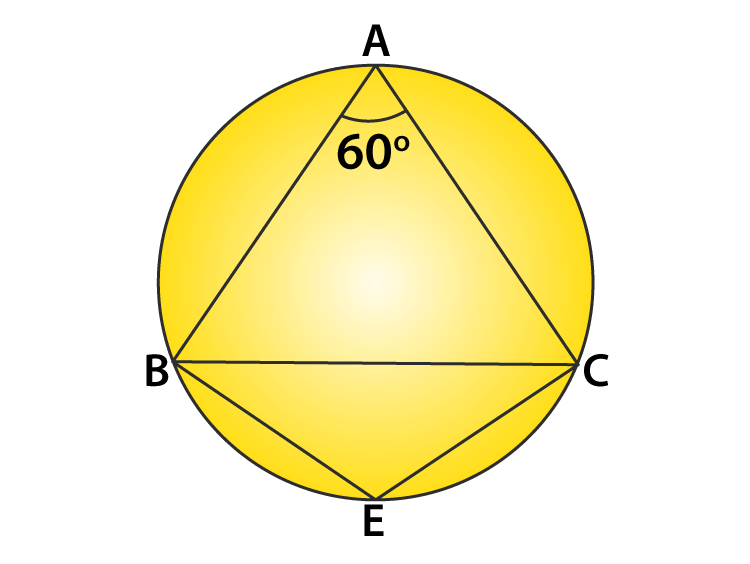Solution:

ΔABC is an equilateral triangle. (Given)

Each angle of an equilateral triangle is 60 degrees.

∠BAC + ∠BEC = 180o (Opposite angles of quadrilateral)

60o + ∠BEC = 180 o

∠BEC = 180 o – 60 o

∠BEC = 120 o

Question 2: In figure, Δ PQR is an isosceles triangle with PQ = PR and m∠PQR=35°. Find m∠QSR and m∠QTR.Solution:

Given: ΔPQR is an isosceles triangle with PQ = PR and m∠PQR = 35°

In ΔPQR:

∠PQR = ∠PRQ = 35o (Angle opposite to equal sides)

Again, by angle sum property

∠P + ∠Q + ∠R = 180 o

∠P + 35 o + 35 o = 180 o

∠P + 70 o = 180 o

∠P = 180 o – 70 o

∠P = 110 o

Now, in quadrilateral SQTR,

∠QSR + ∠QTR = 180 o (Opposite angles of quadrilateral)

110 o + ∠QTR = 180 o

∠QTR = 70 o

Question 3: In figure, O is the centre of the circle. If ∠BOD = 160o, find the values of x and y.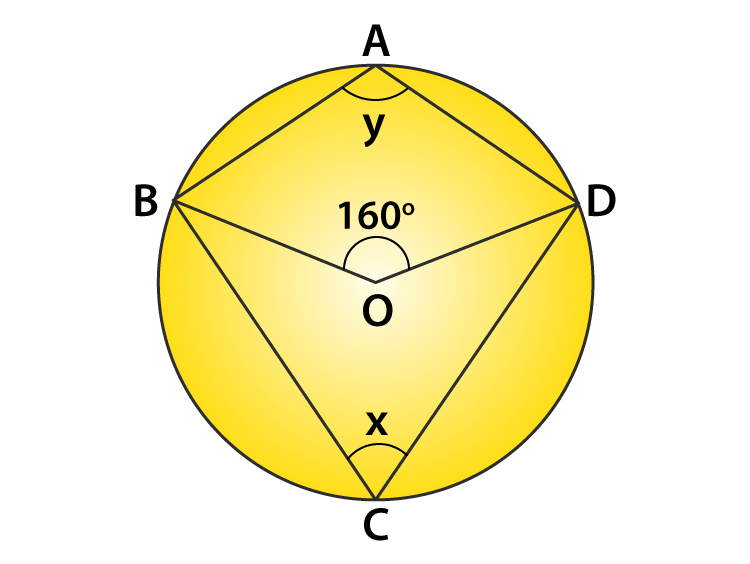Solution:

From figure: ∠BOD = 160 o

By degree measure theorem: ∠BOD = 2 ∠BCD

160 o = 2x

or x = 80 o

Now, in quadrilateral ABCD,

∠BAD + ∠BCD = 180 o (Opposite angles of Cyclic quadrilateral)

y + x = 180 o

Putting value of x,

y + 80 o = 180 o

y = 100 o

Answer: x = 80 o and y = 100 o.

Question 4: In figure, ABCD is a cyclic quadrilateral. If ∠BCD = 100o and ∠ABD = 70o, find ∠ADB.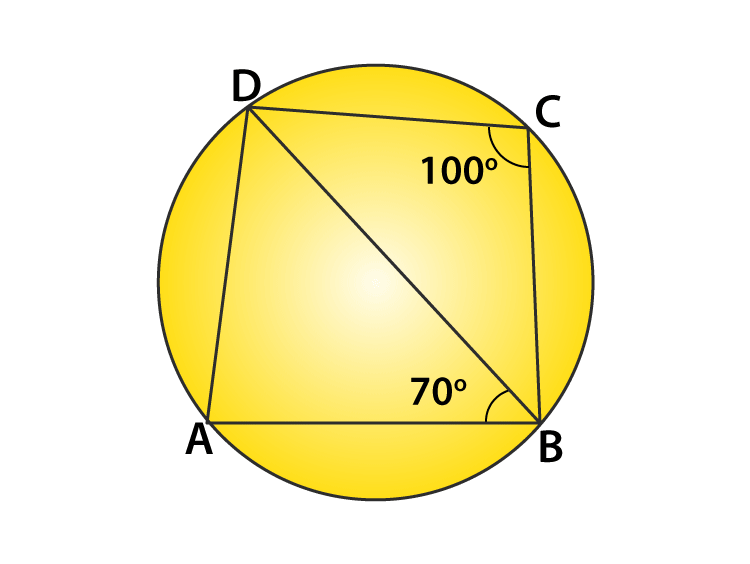Solution:

From figure:

∠DCB + ∠BAD = 180o (Opposite angles of Cyclic quadrilateral)

100 o + ∠BAD = 180o

By angle sum property: ∠ADB + ∠DAB + ∠ABD = 180 o

∠ADB + 80o + 70 o = 180 o

Question 5: If ABCD is a cyclic quadrilateral in which AD||BC (figure). Prove that ∠B = ∠C.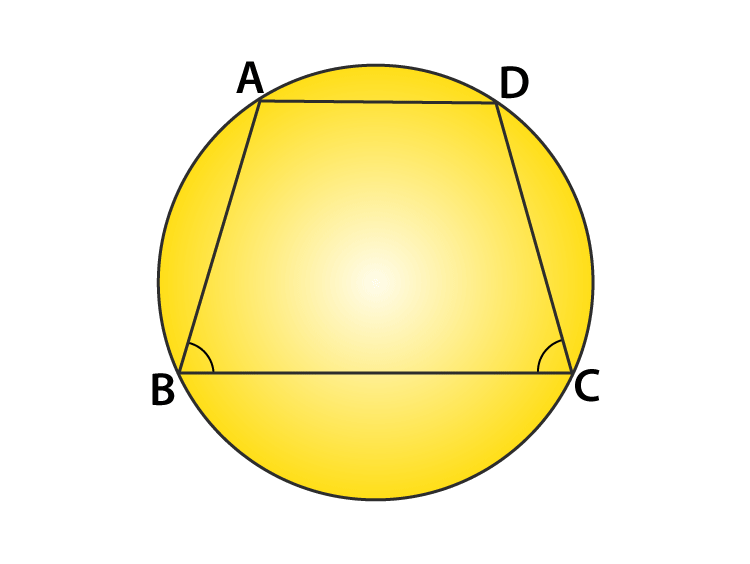Solution:

Given: ABCD is a cyclic quadrilateral with AD ‖ BC

=> ∠A + ∠C = 180o ………(1)

[Opposite angles of cyclic quadrilateral]

and ∠A + ∠B = 180o ………(2)

[Co-interior angles]

Form (1) and (2), we have

∠B = ∠C

Hence proved.

Question 6: In figure, O is the centre of the circle. Find ∠CBD.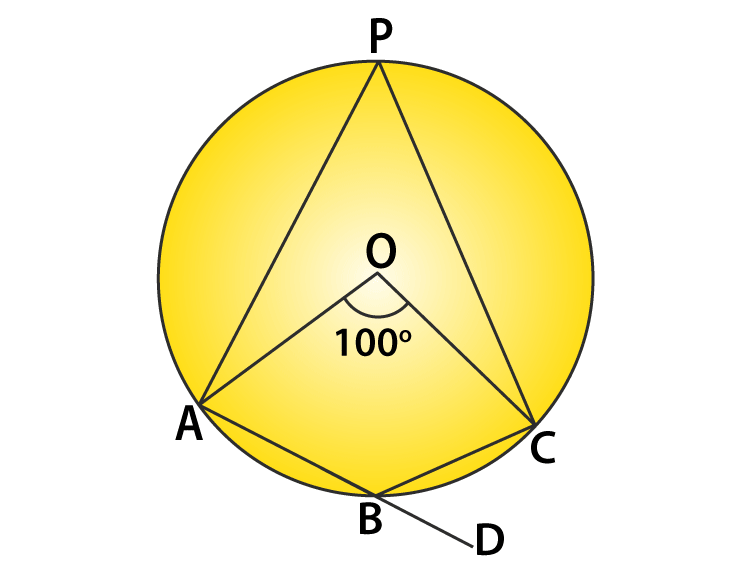Solution:

Given: ∠BOC = 100o

By degree measure theorem: ∠AOC = 2 ∠APC

100 o = 2 ∠APC

or ∠APC = 50 o

Again,

∠APC + ∠ABC = 180 o (Opposite angles of a cyclic quadrilateral)

50o + ∠ABC = 180 o

or ∠ABC = 130 o

Now, ∠ABC + ∠CBD = 180 o (Linear pair)

130o + ∠CBD = 180 o

or ∠CBD = 50 o

Question 7: In figure, AB and CD are diameters of a circle with centre O. If ∠OBD = 500, find ∠AOC.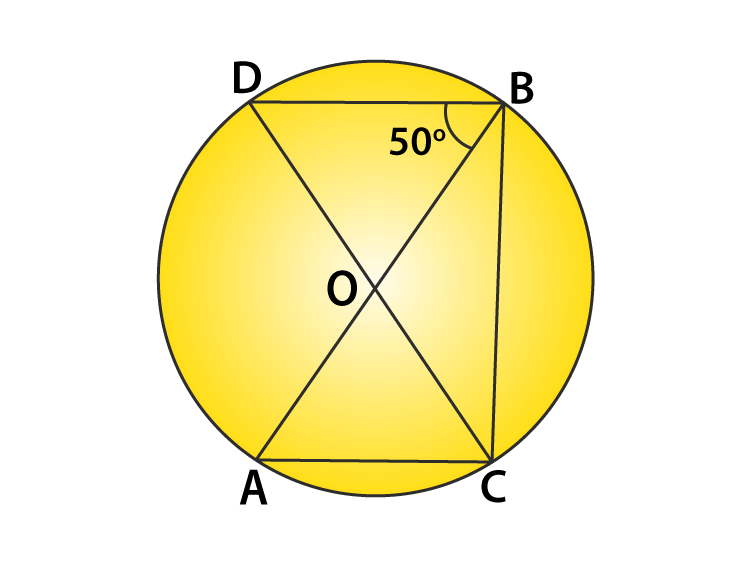Solution:

Given: ∠OBD = 500

Here, AB and CD are the diameters of the circles with centre O.

∠DBC = 900 ….(i)

[Angle in the semi-circle]

Also, ∠DBC = 500 + ∠OBC

900 = 500 + ∠OBC

or ∠OBC = 400

Again, By degree measure theorem: ∠AOC = 2 ∠ABC

∠AOC = 2∠OBC = 2 x 400 = 800

Question 8: On a semi-circle with AB as diameter, a point C is taken, so that m(∠CAB) = 300. Find m(∠ACB) and m(∠ABC).

Solution:

Given: m(∠CAB)= 300

To Find: m(∠ACB) and m(∠ABC).

Now,

∠ACB = 900 (Angle in semi-circle)

Now,

In △ABC, by angle sum property: ∠CAB + ∠ACB + ∠ABC = 1800

300 + 900 + ∠ABC = 1800

∠ABC = 600

Answer: ∠ACB = 900 and ∠ABC = 600

Question 9: In a cyclic quadrilateral ABCD if AB||CD and ∠B = 70o , find the remaining angles.

Solution:

A cyclic quadrilateral ABCD with AB||CD and ∠B = 70o.

∠B + ∠C = 180o (Co-interior angle)

700 + ∠C = 1800

∠C = 1100

And,

=> ∠B + ∠D = 1800 (Opposite angles of Cyclic quadrilateral)

700 + ∠D = 1800

∠D = 1100

Again, ∠A + ∠C = 1800 (Opposite angles of cyclic quadrilateral)

∠A + 1100 = 1800

∠A = 700

Answer: ∠A = 700 , ∠C = 1100 and ∠D = 1100

Question 10: In a cyclic quadrilateral ABCD, if m ∠A = 3(m∠C). Find m ∠A.
Solution:

∠A + ∠C = 180o …..(1)

[Opposite angles of cyclic quadrilateral]

Since m ∠A = 3(m∠C) (given)

=> ∠A = 3∠C …(2)

Equation (1) => 3∠C + ∠C = 180 o

or 4∠C = 180o

or ∠C = 45o

From equation (2)

∠A = 3 x 45o = 135o

Question 11: In figure, O is the centre of the circle ∠DAB = 50°. Calculate the values of x and y.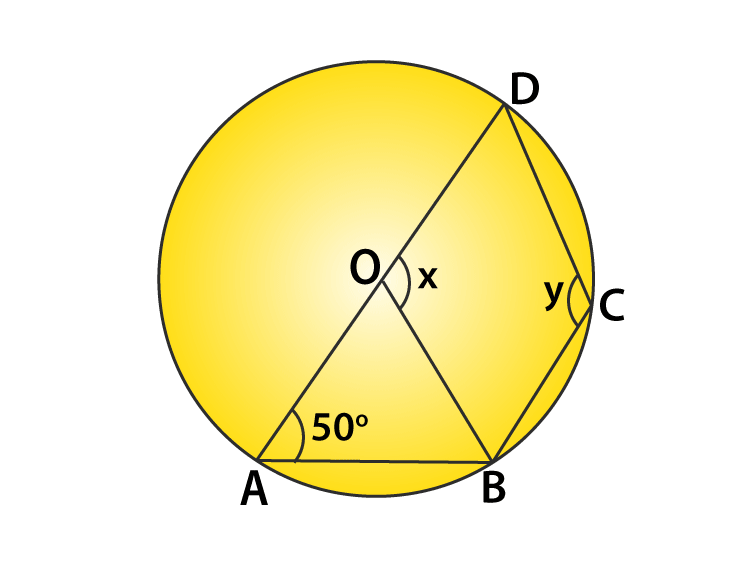Solution:

Given : ∠DAB = 50o

By degree measure theorem: ∠BOD = 2 ∠BAD

so, x = 2( 500) = 1000

Since, ABCD is a cyclic quadrilateral, we have

∠A + ∠C = 1800

500 + y = 1800

y = 1300

### Exercise VSAQs Page No: 16.89

Question 1: In figure, two circles intersect at A and B. The centre of the smaller circle is O and it lies on the circumference of the larger circle. If ∠APB = 70°, find ∠ACB.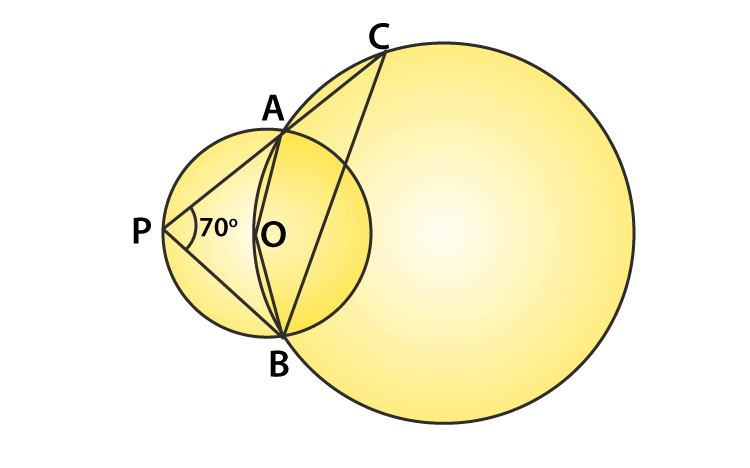Solution:

By degree measure theorem: ∠AOB = 2 ∠APB

so, ∠AOB = 2 × 70° = 140°

Since AOBC is a cyclic quadrilateral, we have

∠ACB + ∠AOB = 180°

∠ACB + 140° = 180°

∠ACB = 40°

Question 2: In figure, two congruent circles with centres O and O’ intersect at A and B. If ∠AO’B = 50°, then find ∠APB.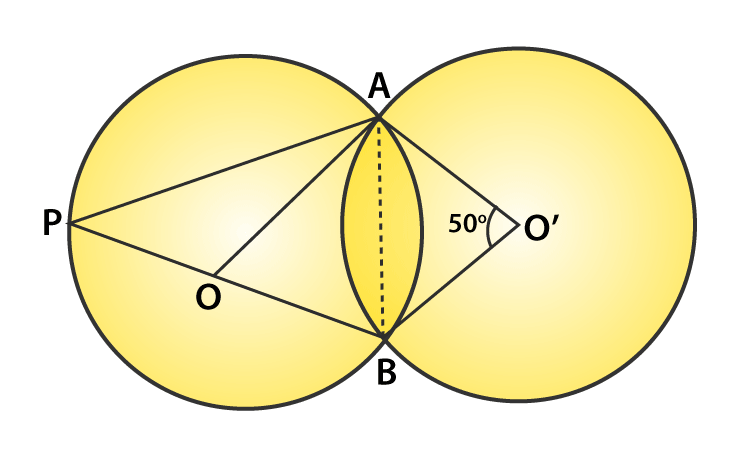Solution:

As we are given that, both the triangle are congruent which means their corresponding angles are equal.

Therefore, ∠AOB = AO’B = 50°

Now, by degree measure theorem, we have

∠APB = ∠AOB/2 = 250

Question 3: In figure, ABCD is a cyclic quadrilateral in which ∠BAD=75°, ∠ABD=58° and ∠ADC=77°, AC and BD intersect at P. Then, find ∠DPC.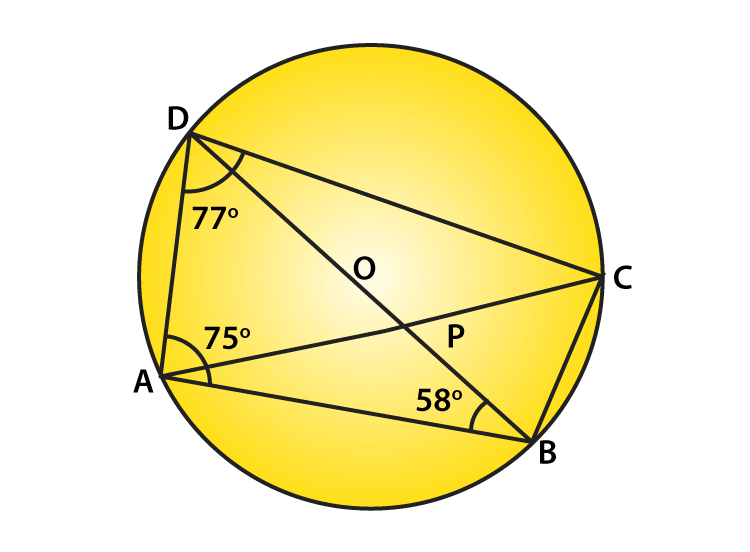Solution:

∠DBA = ∠DCA = 580 …(1)

[Angles in same segment]

ABCD is a cyclic quadrilateral :

Sum of opposite angles = 180 degrees

∠A +∠C = 1800

750 + ∠C = 1800

∠C = 1050

Again, ∠ACB + ∠ACD = 1050

∠ACB + 580 = 1050

or ∠ACB = 470 …(2)

Now, ∠ACB = ∠ADB = 470

[Angles in same segment]

Also, ∠D = 770 (Given)

Again From figure, ∠BDC + ∠ADB = 770

∠BDC + 470 = 770

∠BDC = 300

In triangle DPC

∠PDC + ∠DCP + ∠DPC = 1800

300 + 580 + ∠DPC = 1800

or ∠DPC = 92

Question 4: In figure, if ∠AOB = 80° and ∠ABC=30°, then find ∠CAO.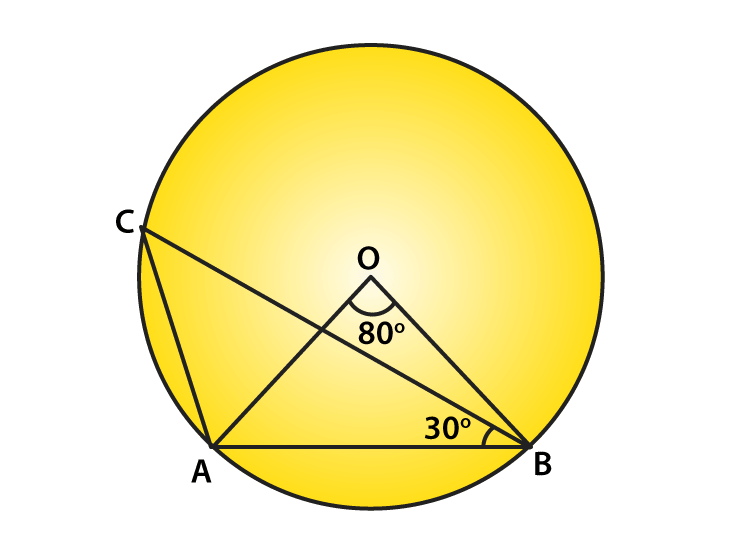Solution:

Given: ∠AOB = 800 and ∠ABC = 300

To find: ∠CAO

Join OC.

Central angle subtended by arc AC = ∠COA

then ∠COA = 2 x ∠ABC = 2 x 300 = 600 …(1)

In triangle OCA,

OC = OA

∠OCA = ∠CAO …(2)

[Angle opposite to equal sides]

In triangle COA,

∠OCA + ∠CAO + ∠COA = 1800

From (1) and (2), we get

2∠CAO + 600 = 1800

∠CAO = 600

## RD Sharma Solutions for Class 9 Maths Chapter 16 Circles

In the 16th Chapter of Class 9, RD Sharma Solutions students will study important concepts. Some of the concepts are listed below:

• Circle Introduction
• Position of a point with respect to a circle
• Circular Disc
• Concentric Circles
• Degree measure of an arc
• Chord and segment of a circle
• Congruence of circles and arcs

## Frequently Asked Questions on RD Sharma Solutions for Class 9 Maths Chapter 16

### Why should we refer to RD Sharma Solutions for Class 9 Maths Chapter 16?

RD Sharma Solutions for Class 9 Maths Chapter 16 is the best guide that offers complete and quality information of the concepts vital for examinations. The solutions are developed by expert faculty in an easy to remember format, which further helps students to understand the concepts effectively and remember the answers. Hence, teachers recommend students to download the solutions PDF to score good marks in exams.

### How BYJU’S RD Sharma Solutions for Class 9 Maths Chapter 16 help students to score good marks in CBSE board?

RD Sharma Solutions for Class 9 Maths Chapter 16 provide in-depth knowledge of concepts in a simple and understandable language. Each step is explained in detail format to help students clear their doubts instantly. Thorough practice of these solutions enables students to enhance their skills and procure more marks in their CBSE board.

### What are the topics covered under RD Sharma Solutions for Class 9 Maths Chapter 16?

The concepts covered under RD Sharma Solutions for Class 9 Maths Chapter 16 are as follows:

• Circle Introduction
• Position of a point with respect to a circle
• Circular Disc
• Concentric Circles
• Degree measure of an arc
• Chord and segment of a circle
• Congruence of circles and arcs